# RD Sharma Solutions for Class 8 Maths Chapter 21 Mensuration - II (Volumes and Surface Areas of a Cuboid and a cube)

This chapter mainly deals with volumes and surface areas of a cuboid and a cube. Some applications of these formulae to solve simple problems from everyday life situations will also be discussed. Students having any difficulties in solving problems can utilize RD Sharma Class 8. Solutions here are formulated by our expert faculty team with utmost care to help students understand the concepts clearly. Students can use RD Sharma textbook as reference material for preparing for their board exams. PDF consisting of this chapter solutions can be easily downloaded from the links provided below.

Chapter 21- Mensuration – II (Volumes and Surface Areas of a Cuboid and a cube) contains four exercises and the RD Sharma Class 8 Solutions present in this page provide solutions to the questions present in each exercise. Now, let us have a look at the concepts discussed in this chapter.

• Cuboid.
• Space regions.
• The volume of the space region formed by a body.
• A standard unit of volume.
• The formula for finding the volume of a cuboid.
• The volume of a cube.
• Other standard units of volume.
• Surface areas of a cuboid and a cube.
• The surface area of the walls of a room.
• Miscellaneous problems.

## Download the Pdf of RD Sharma Solutions for Class 8 Maths Chapter 21 Mensuration – II (Volumes and Surface Areas of a Cuboid and a cube)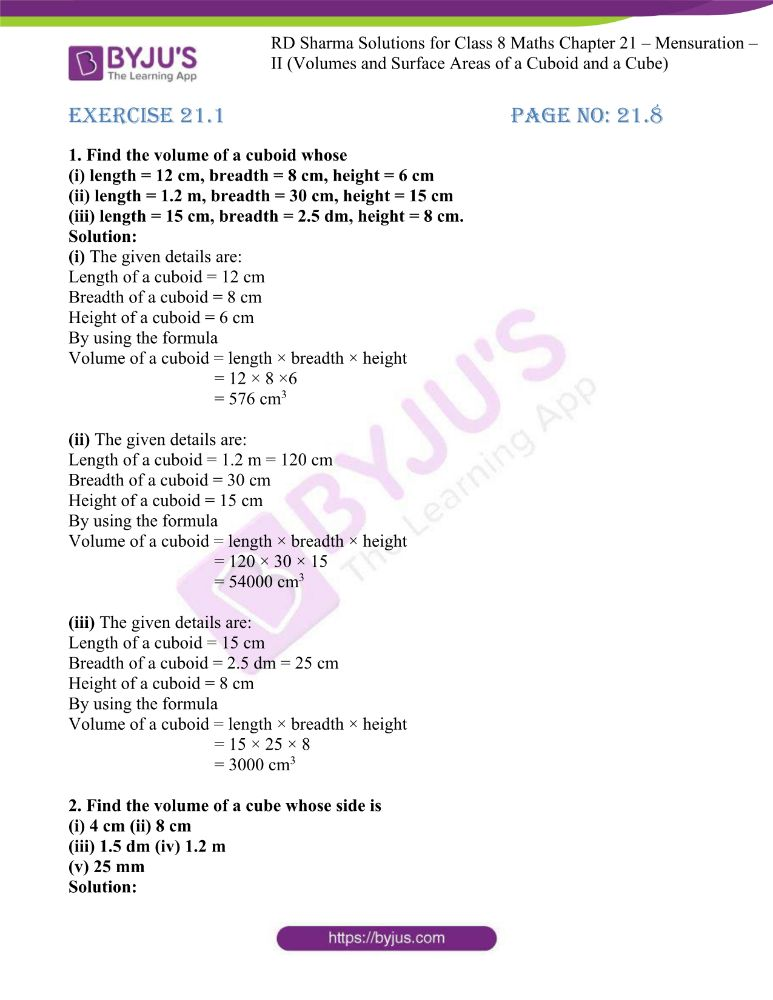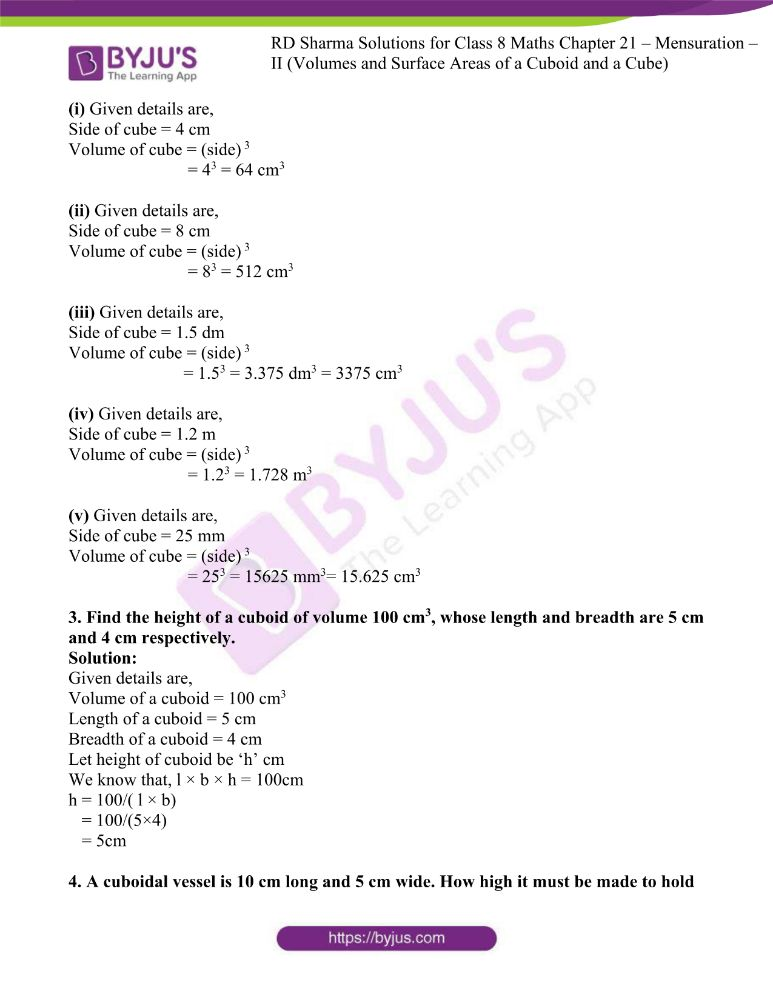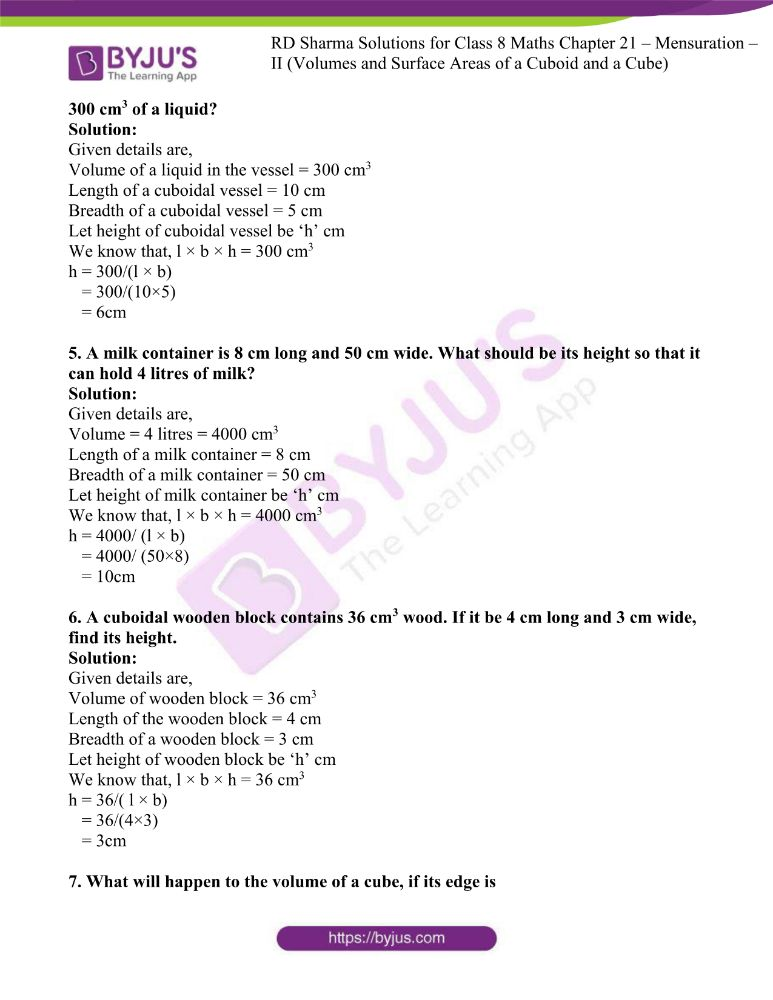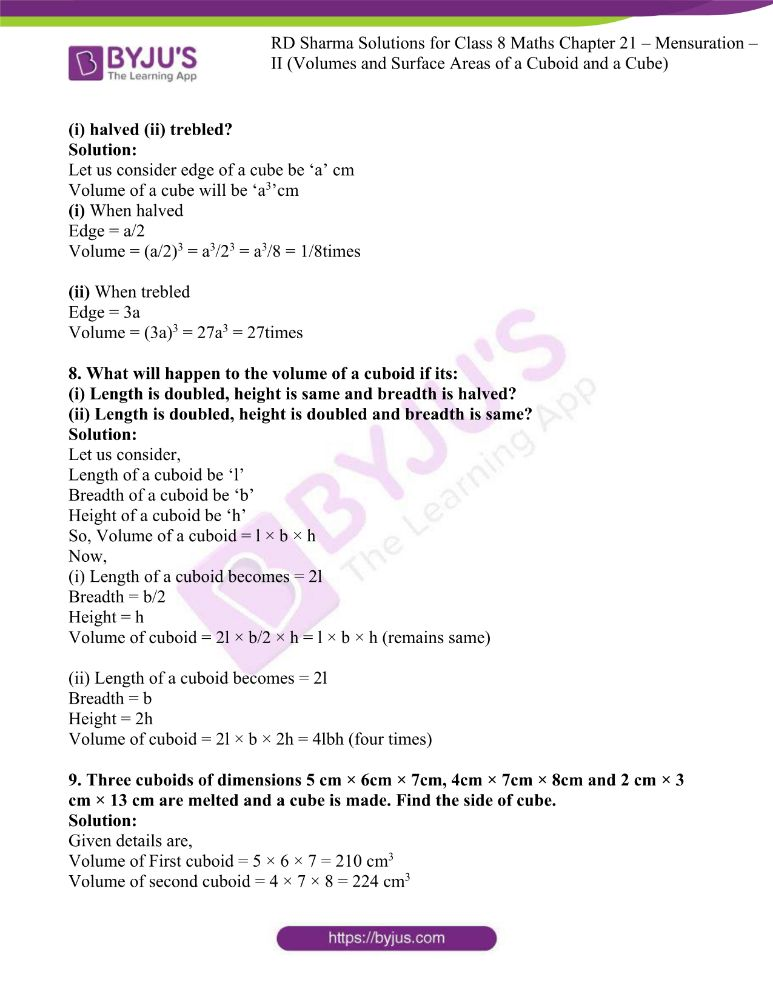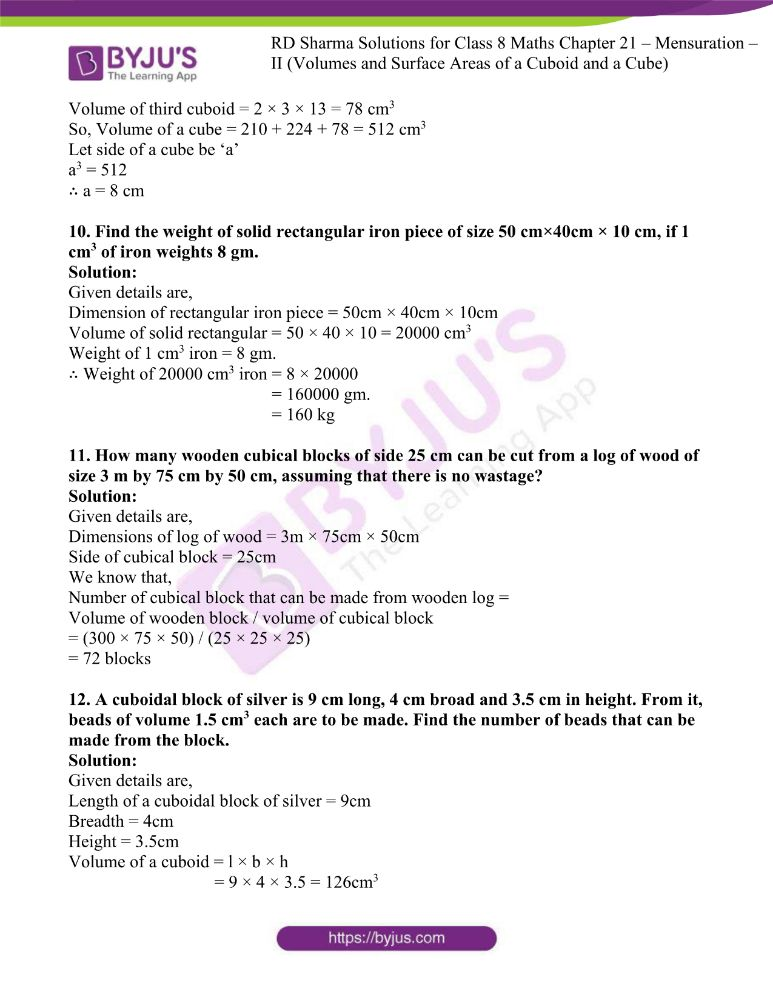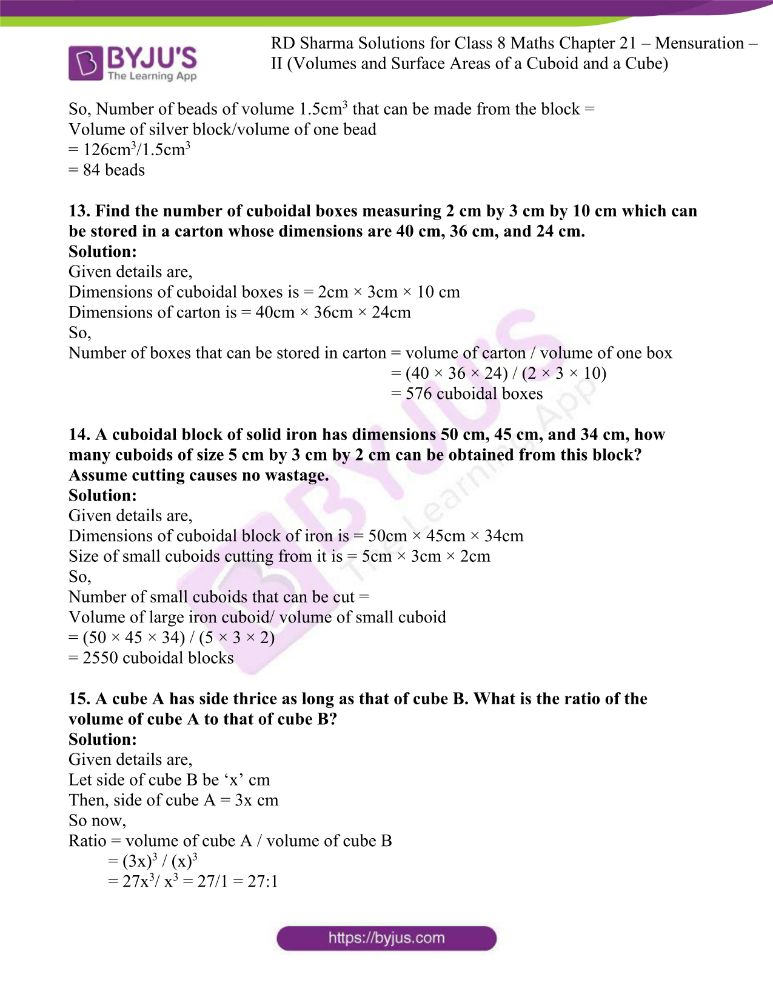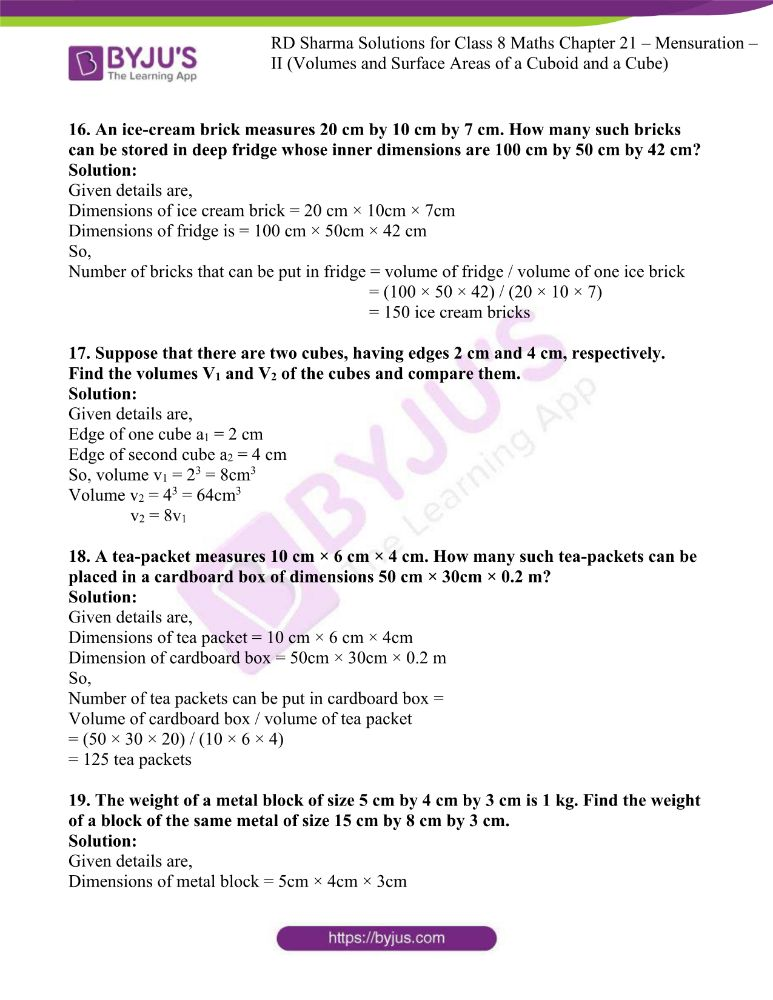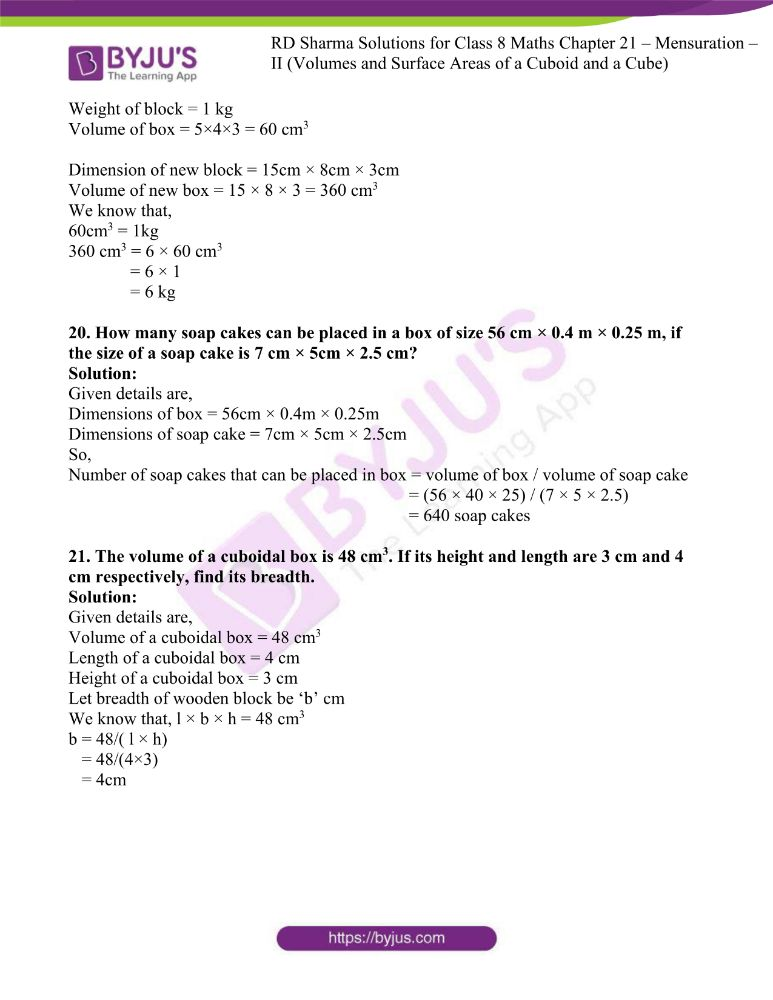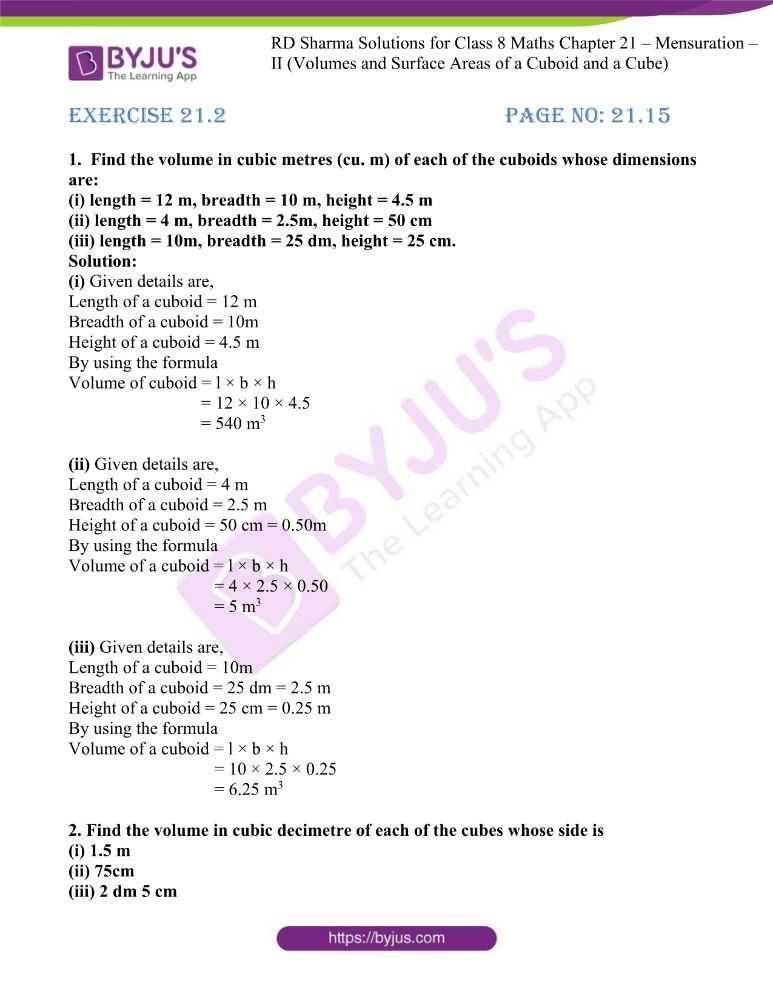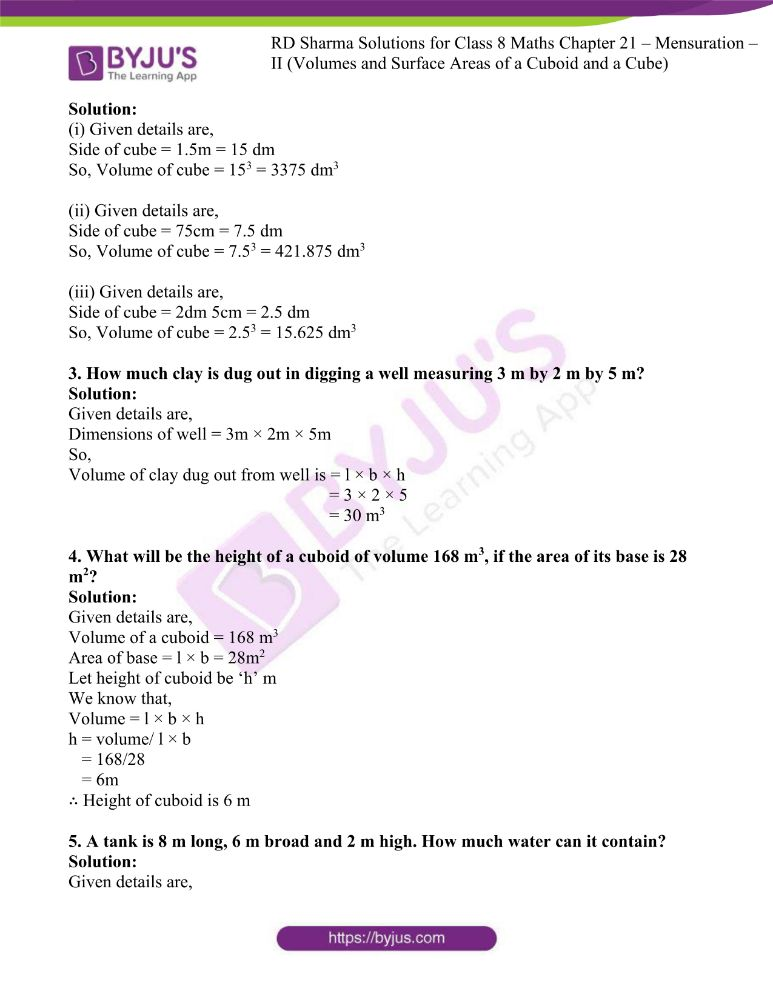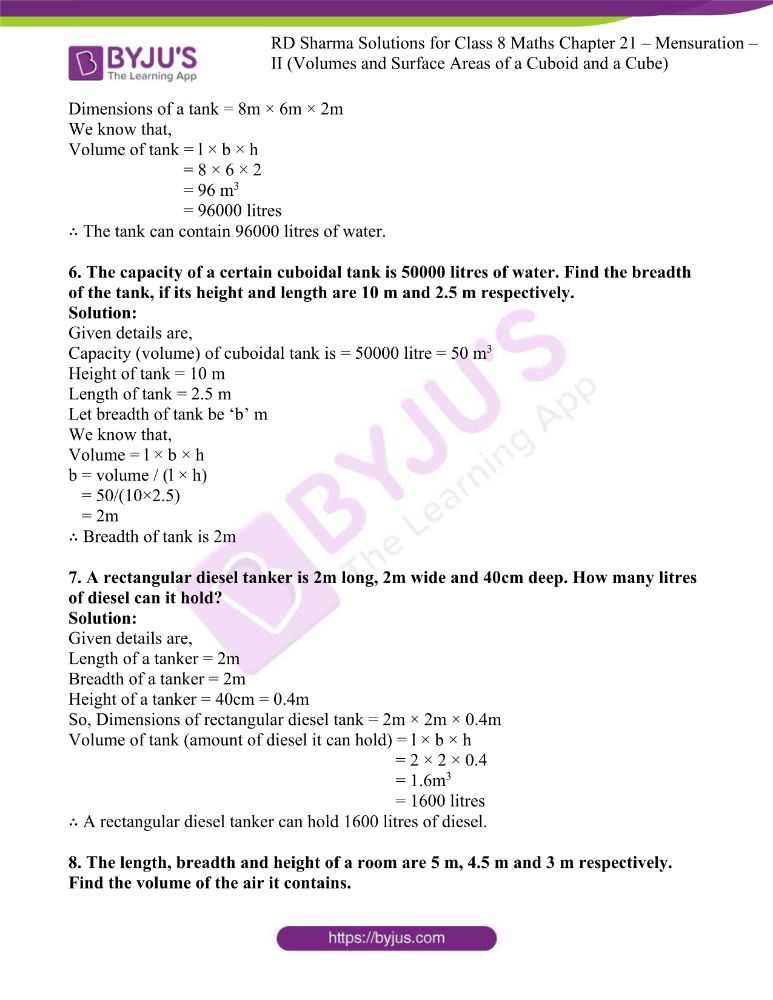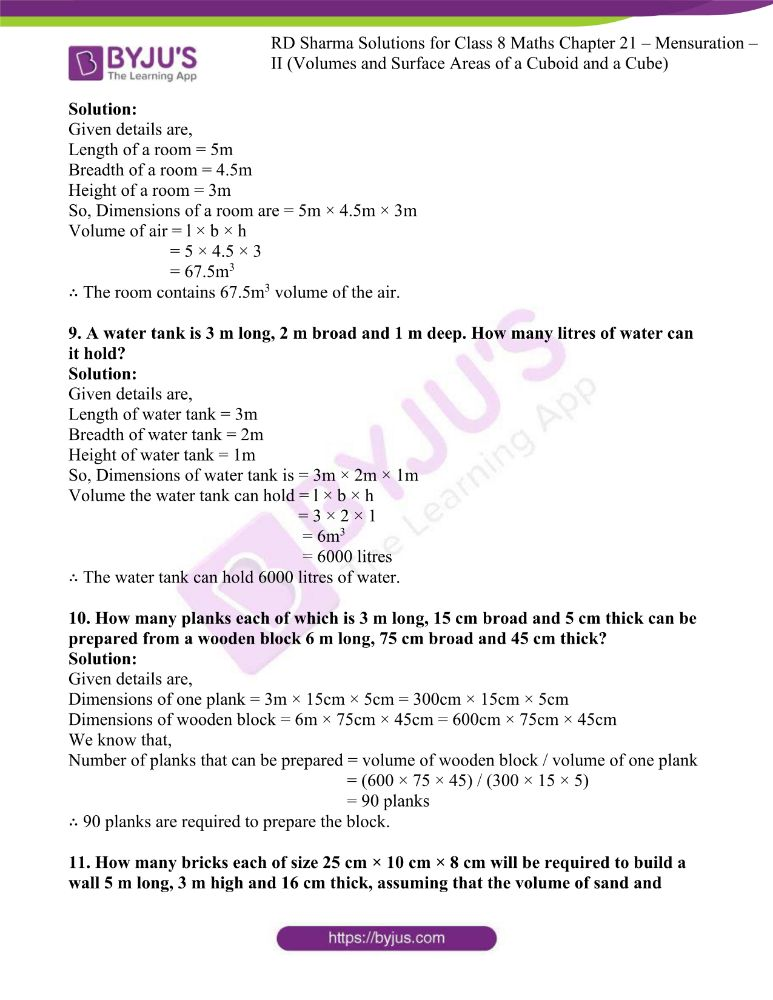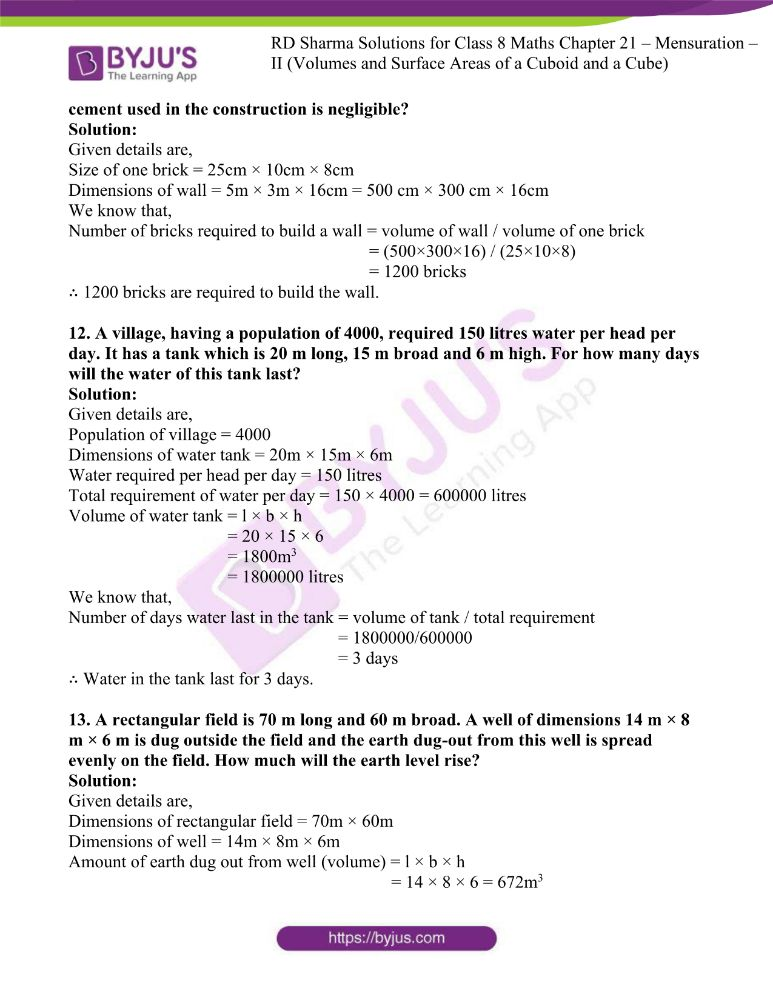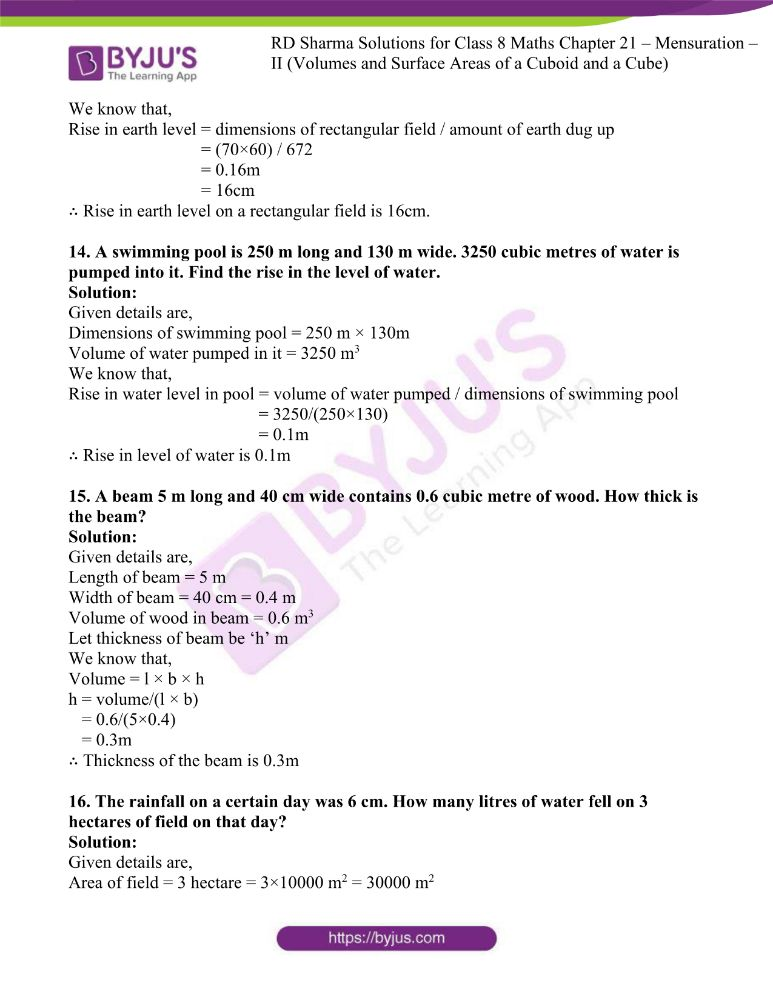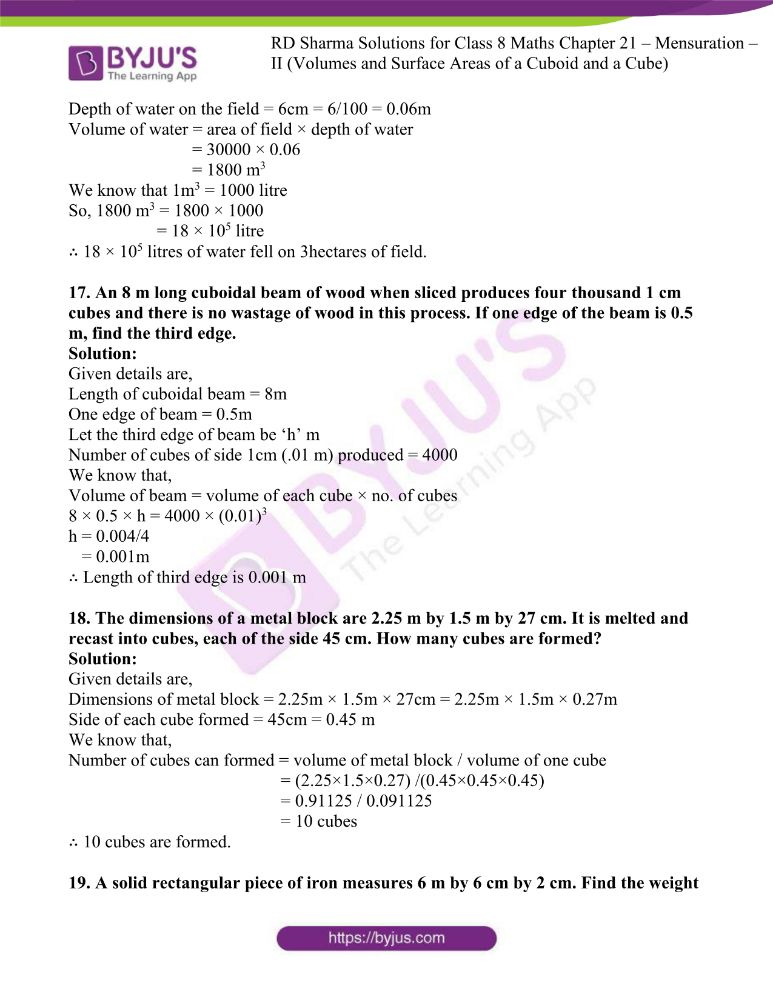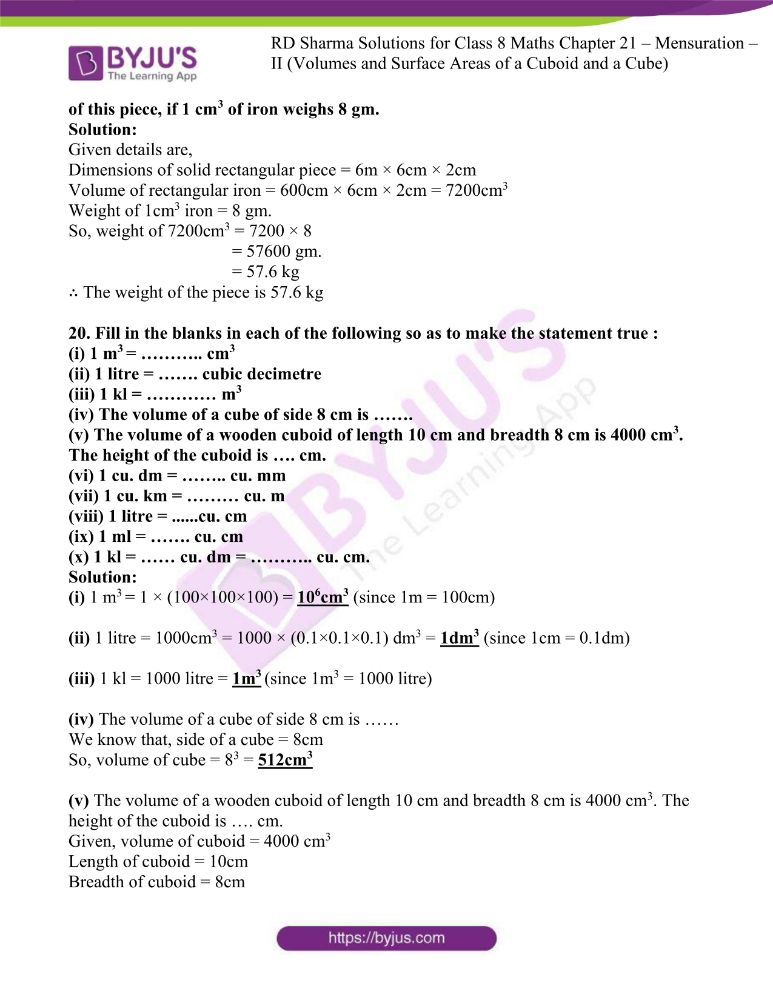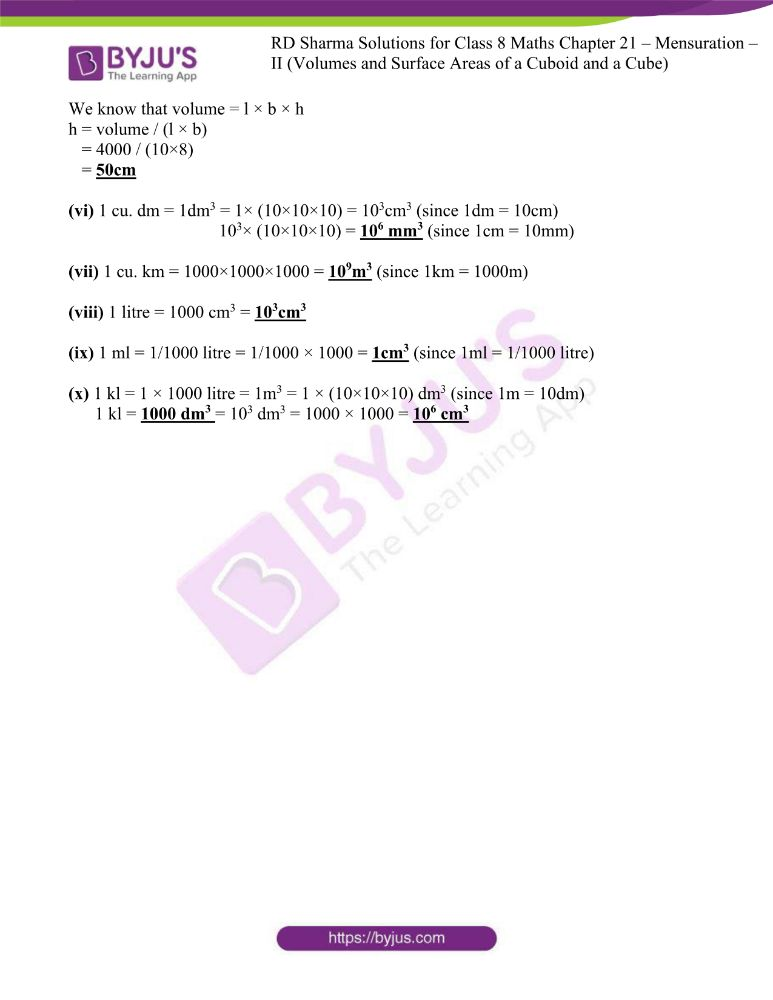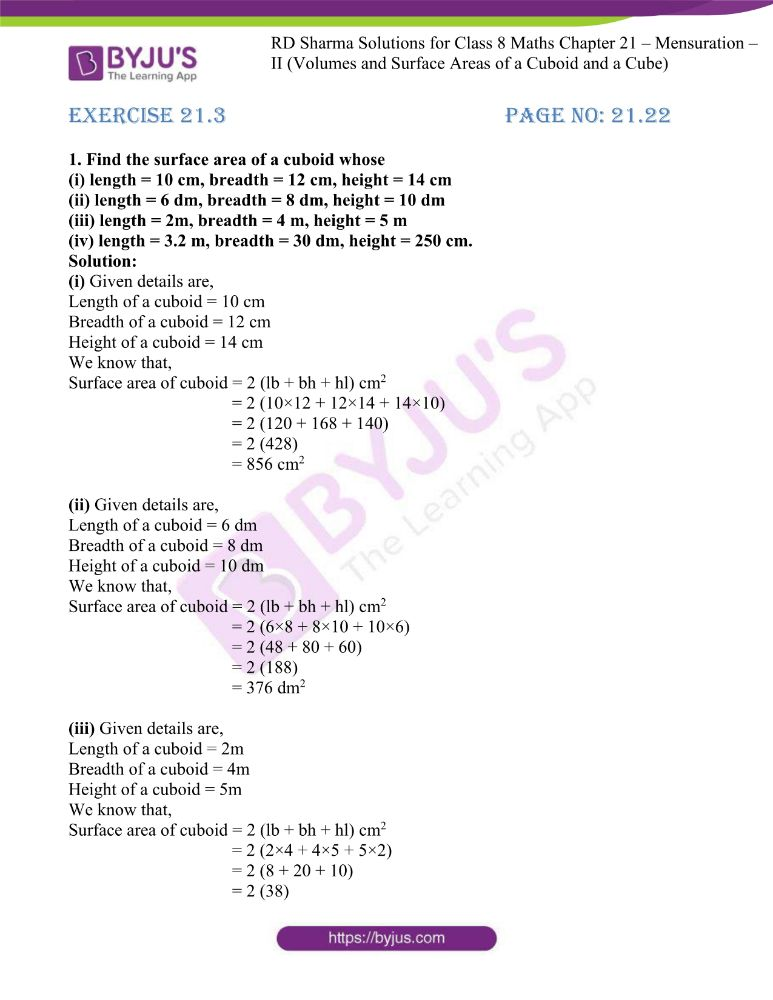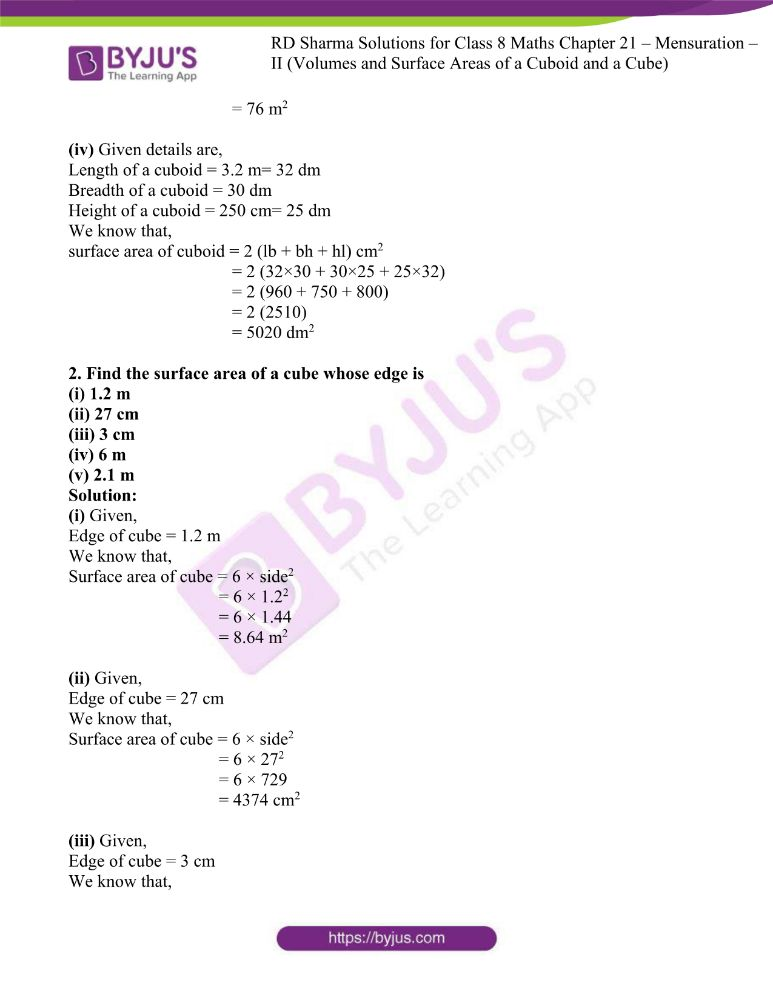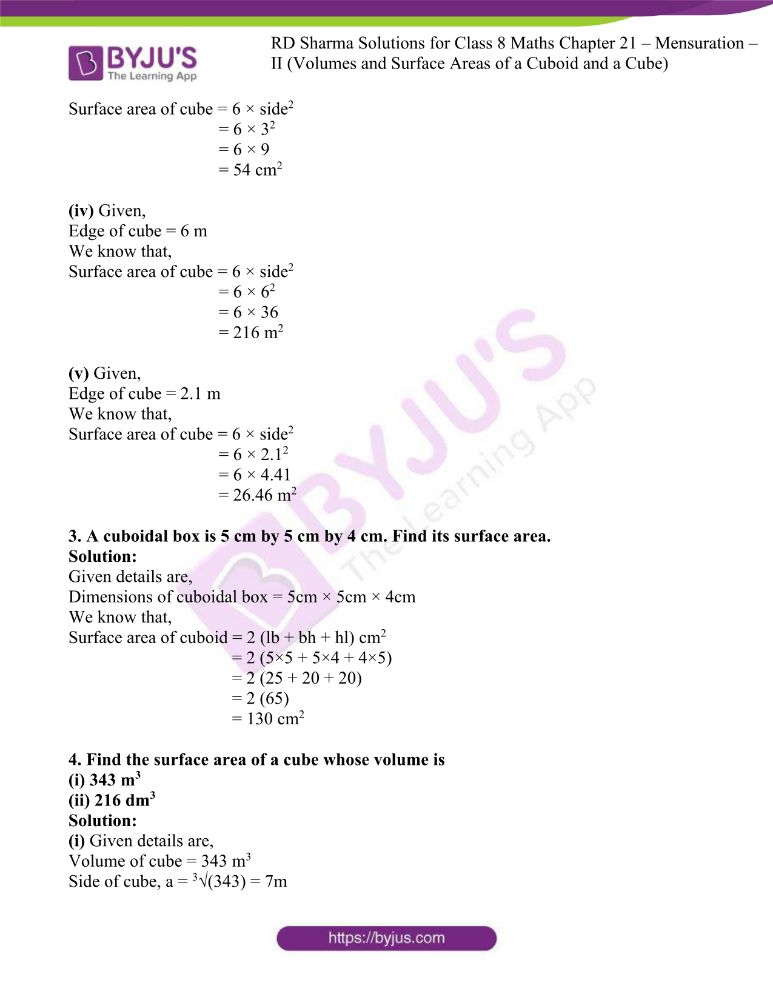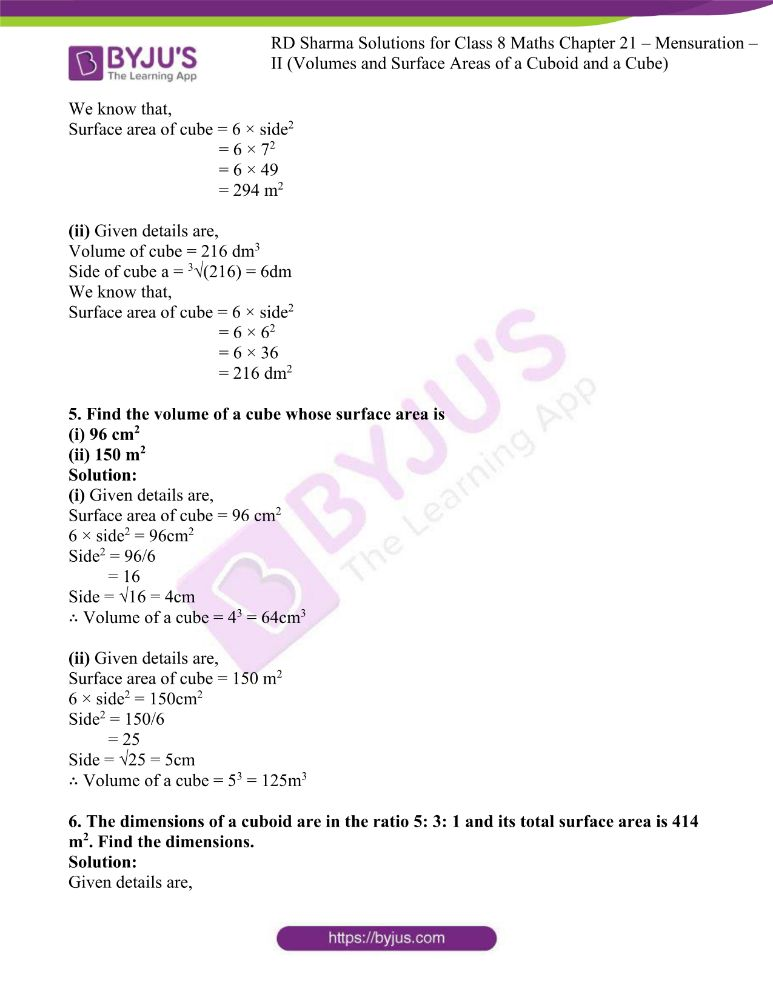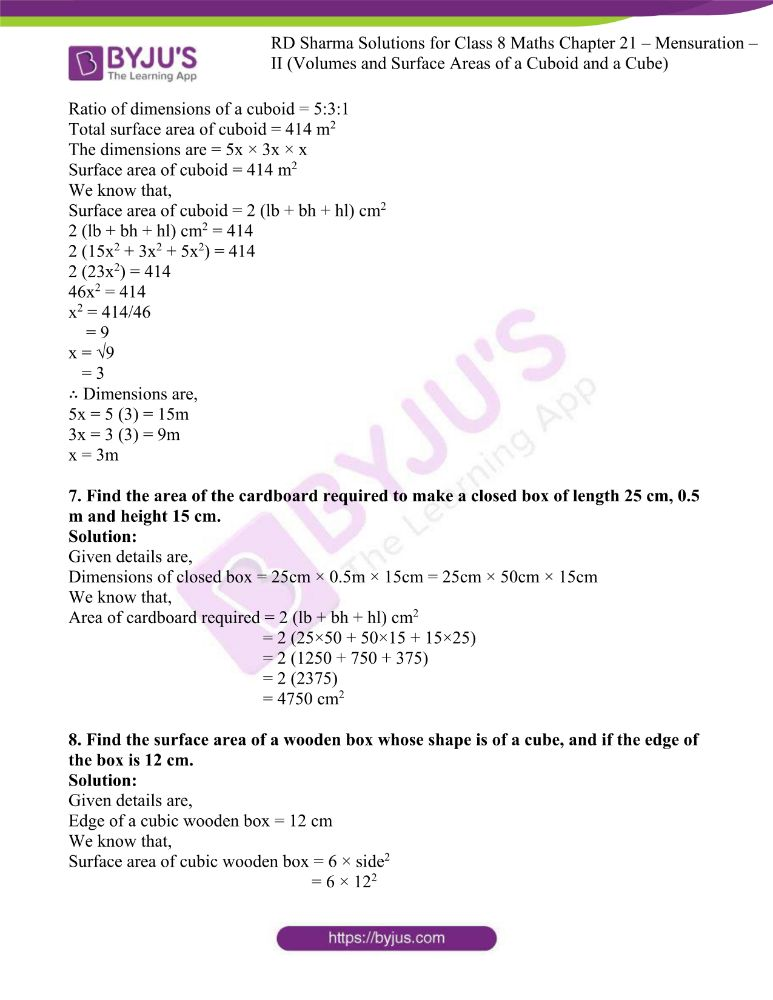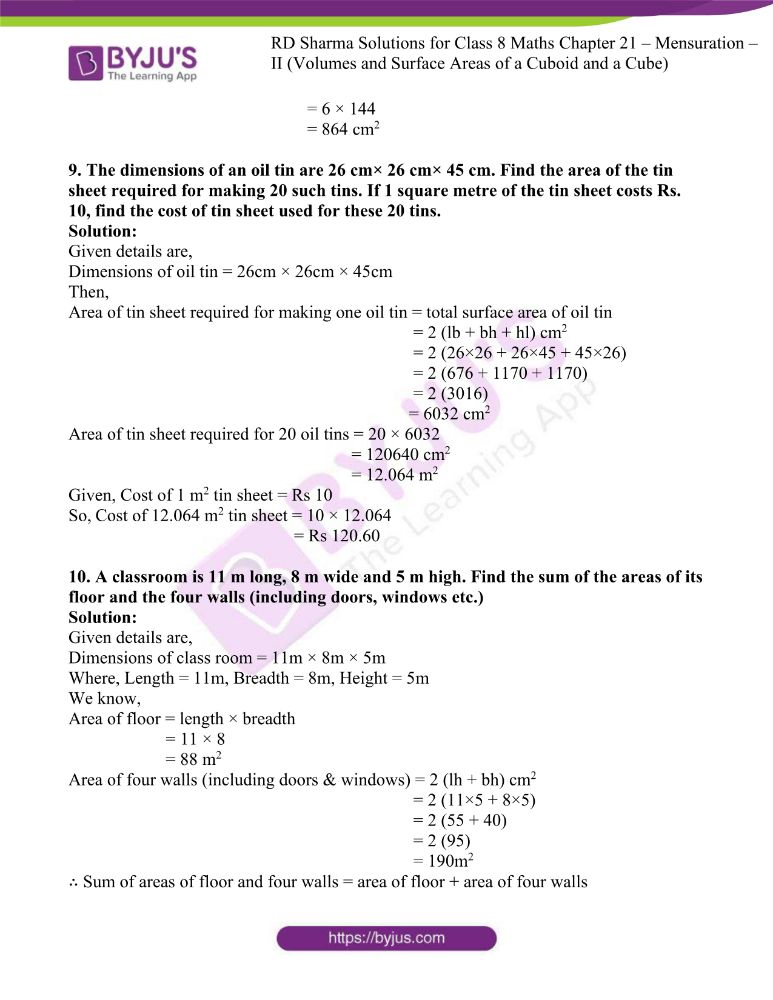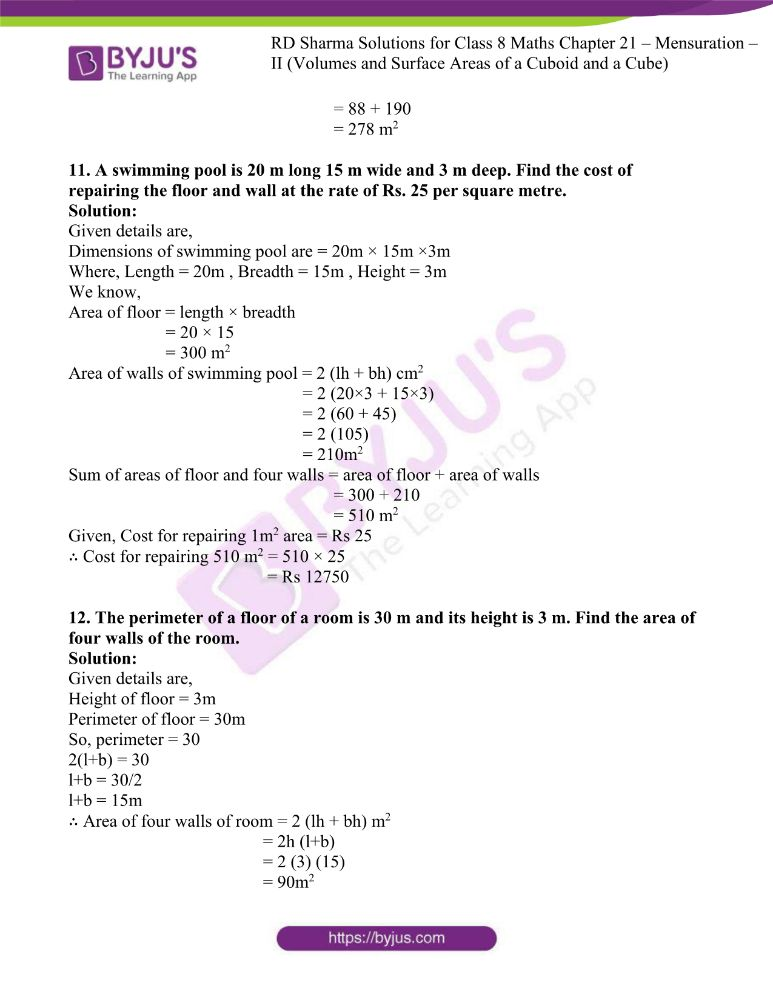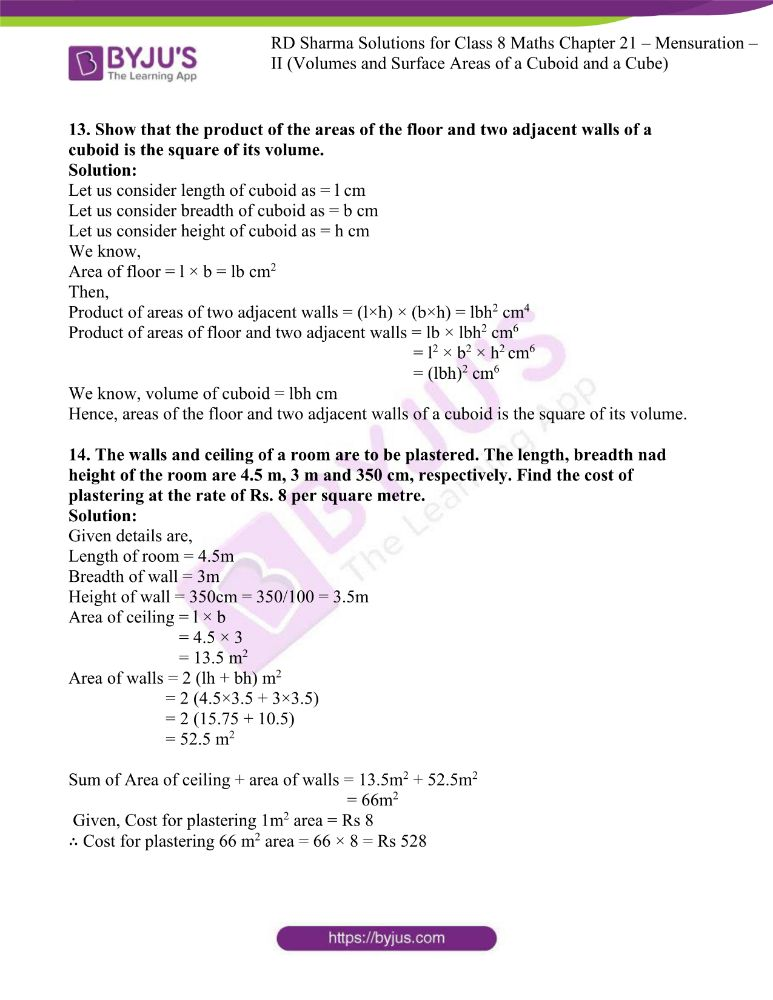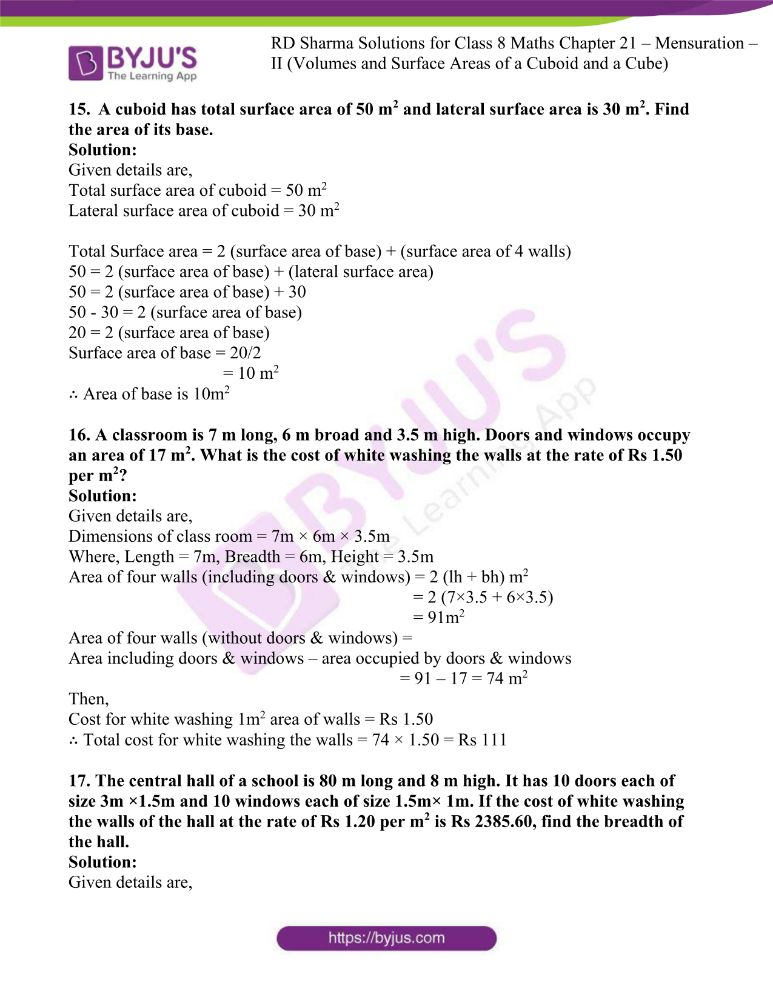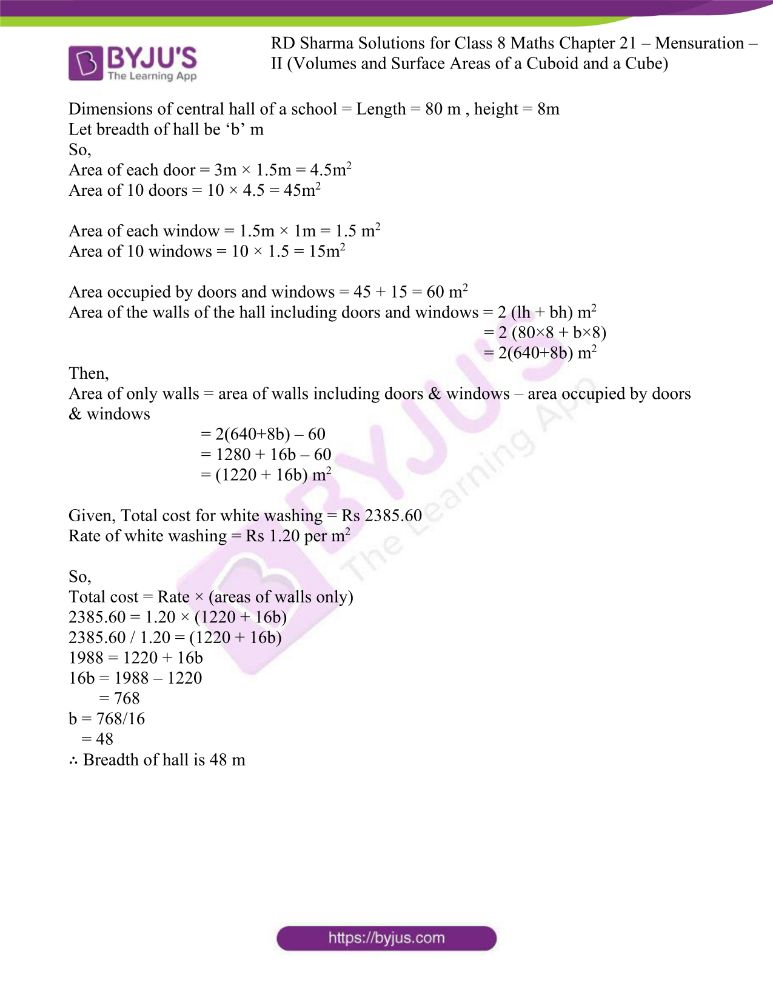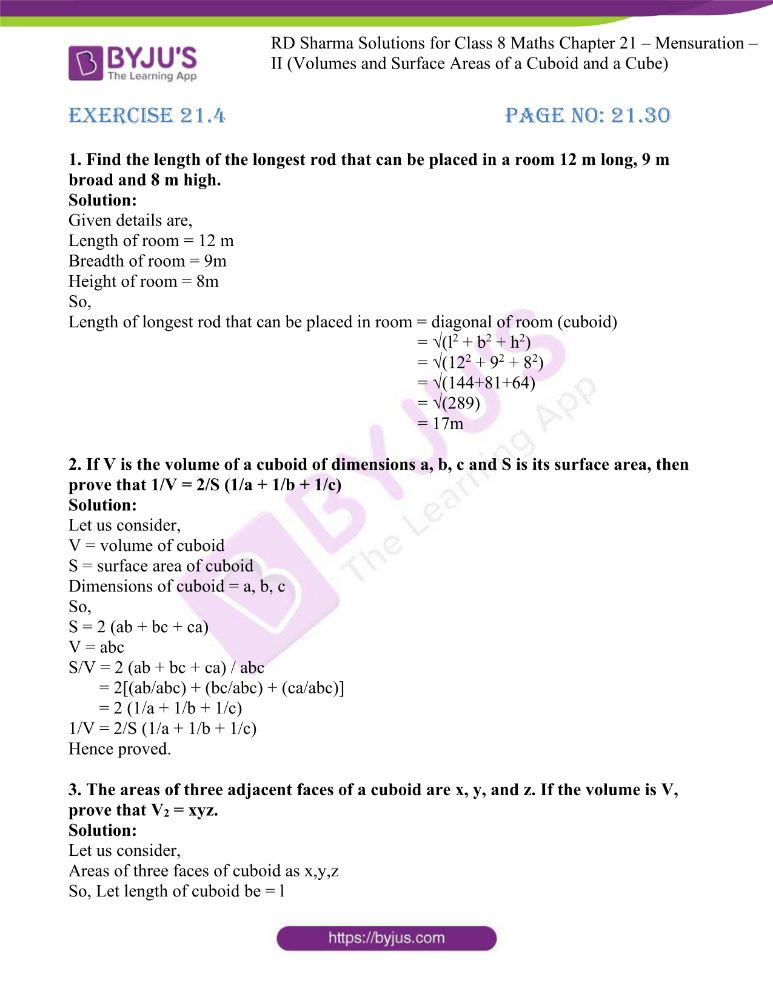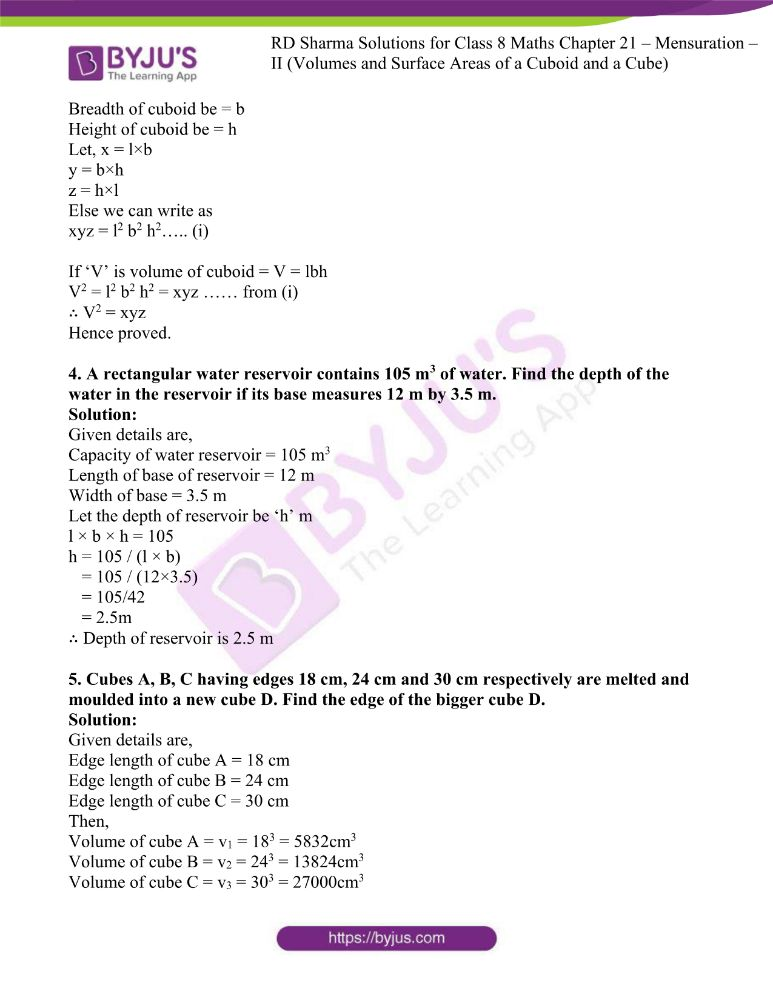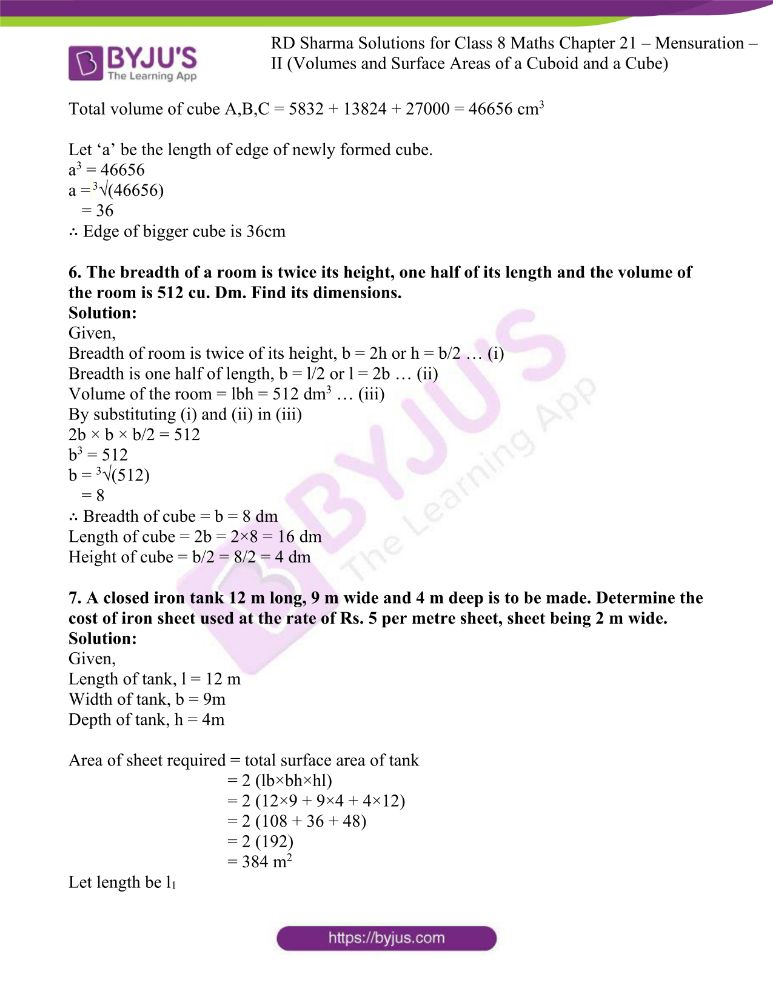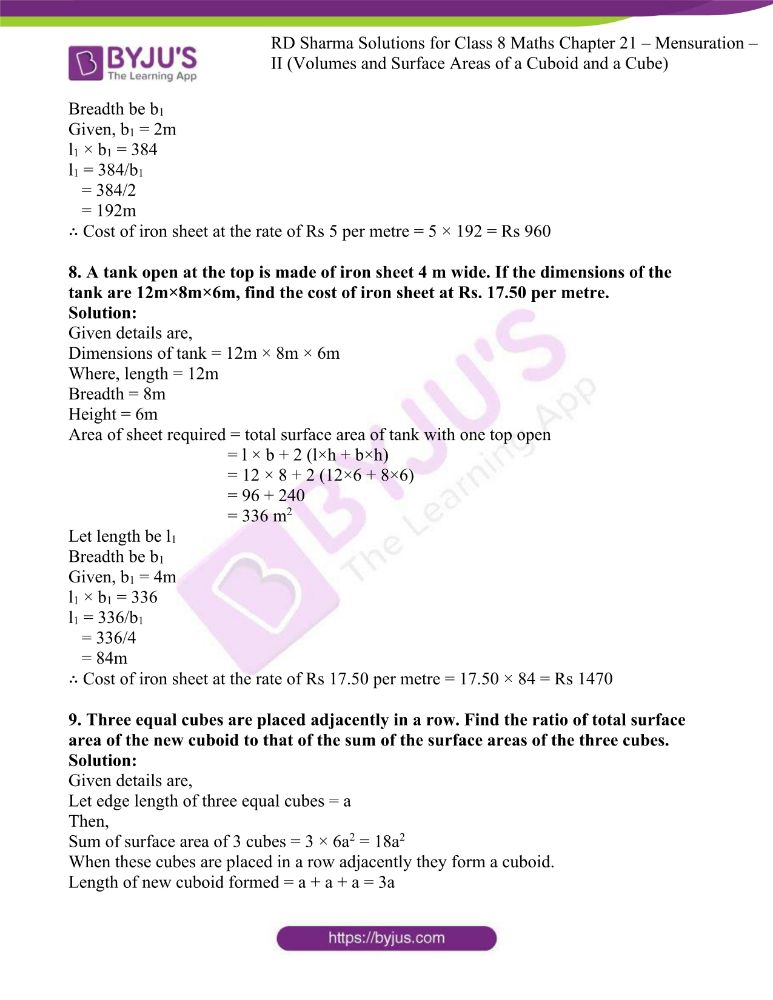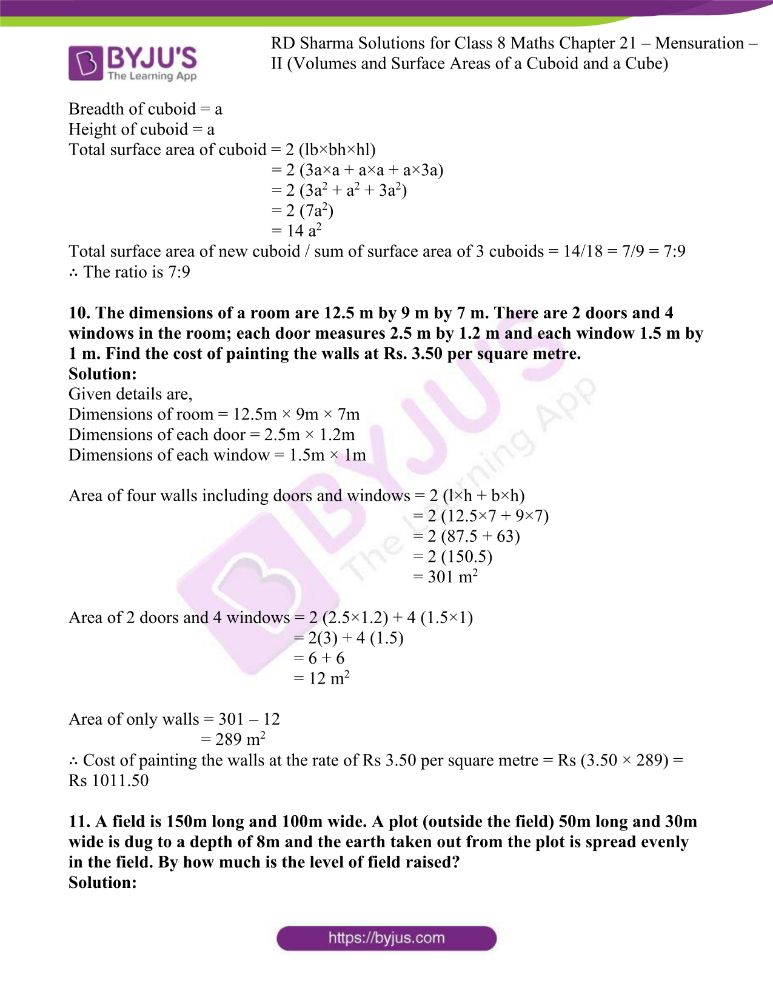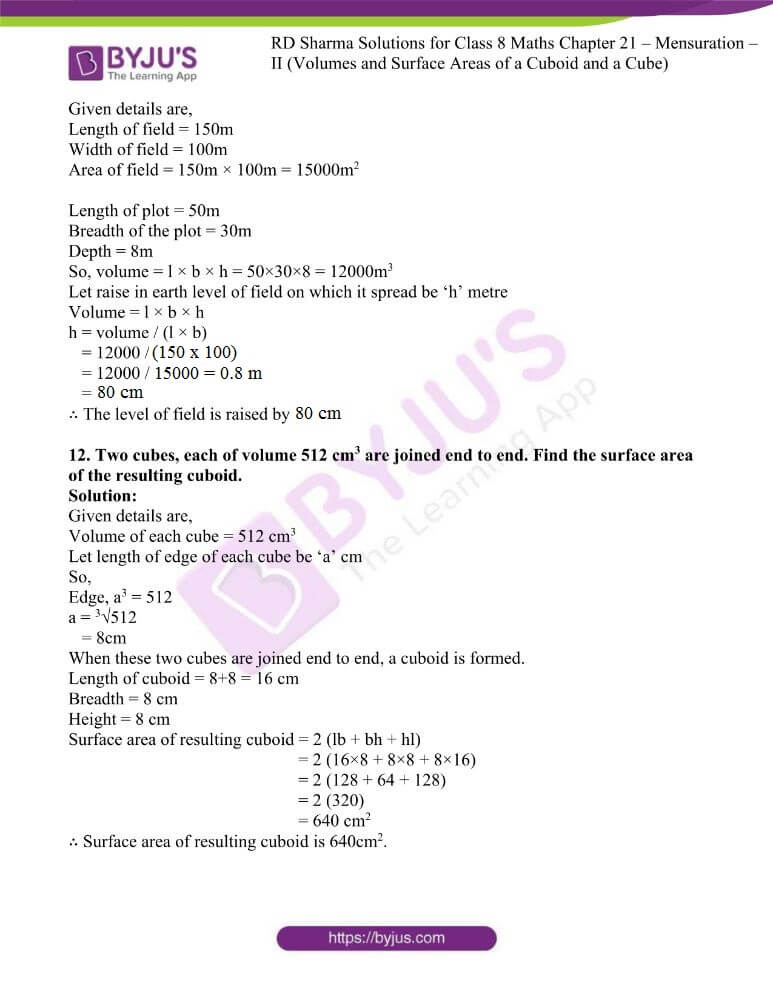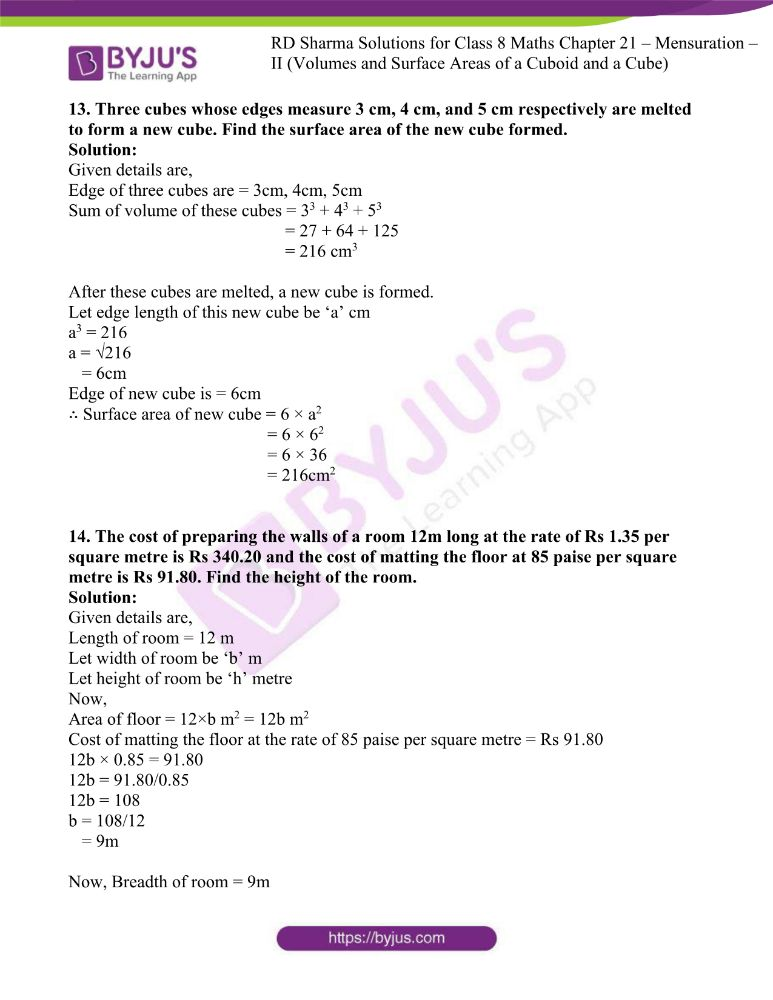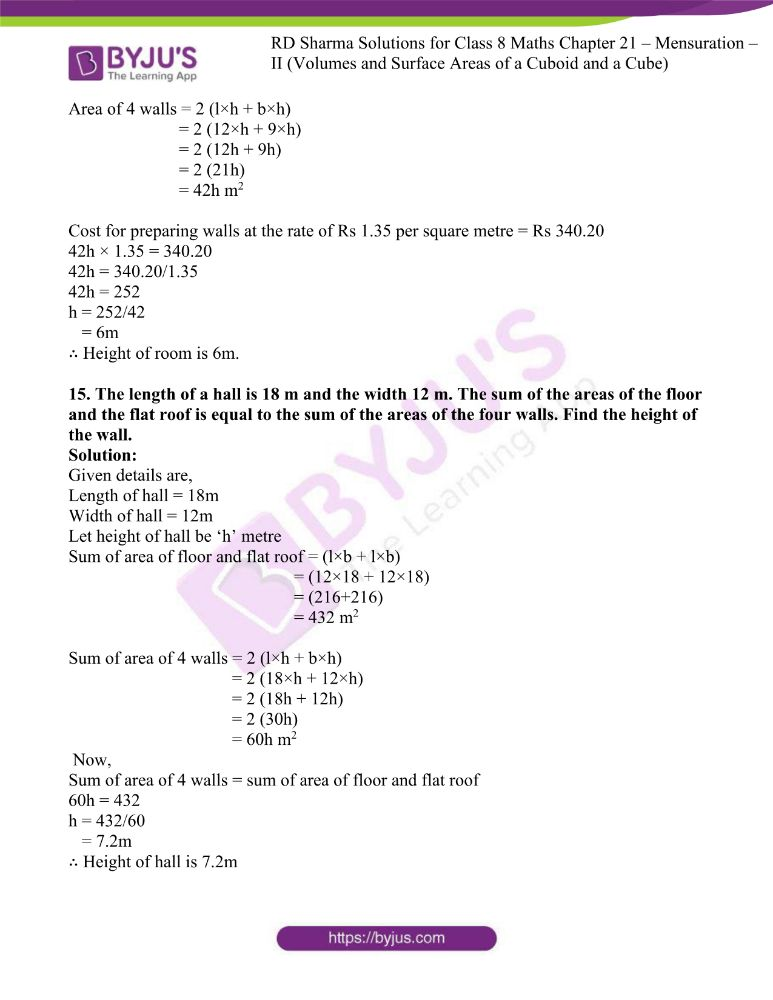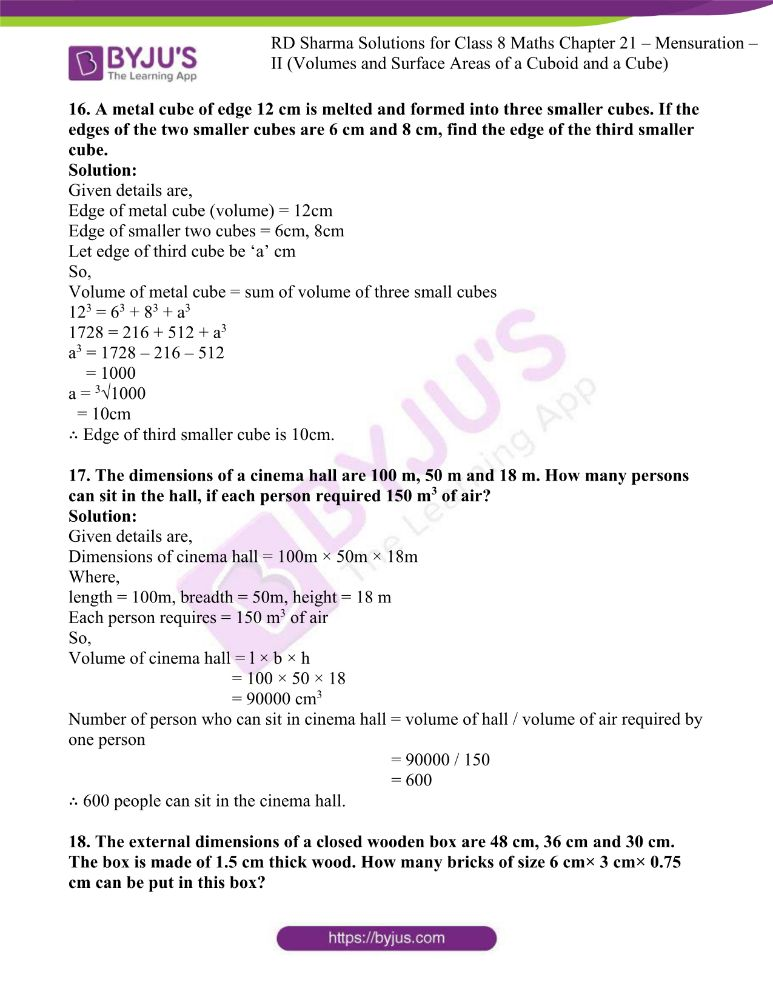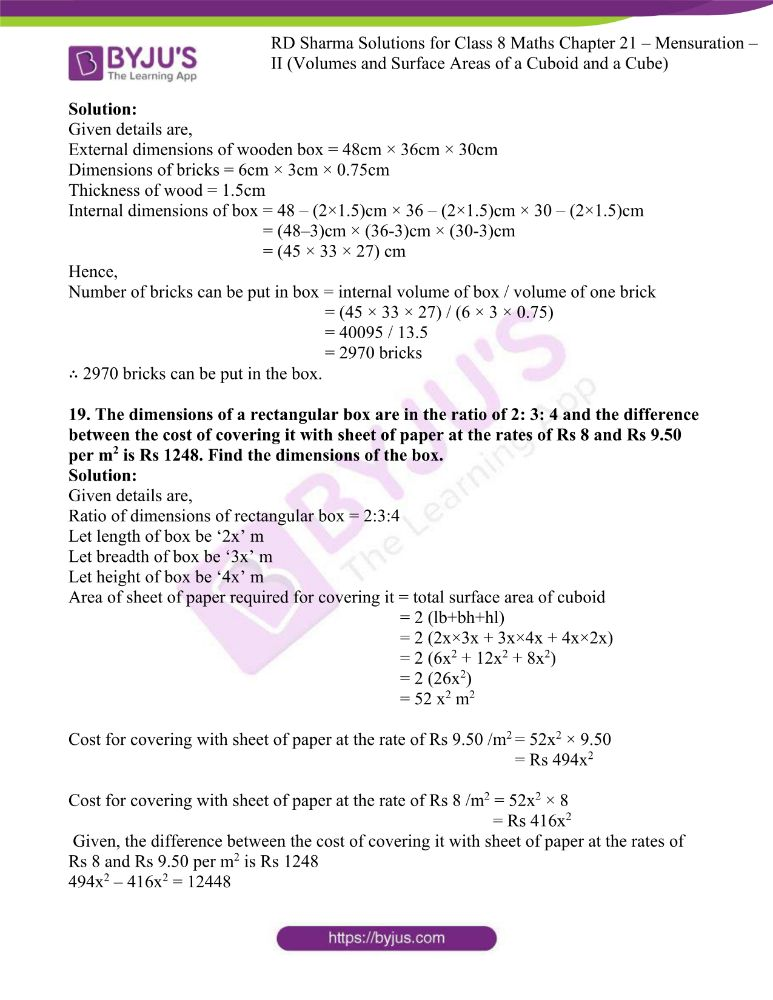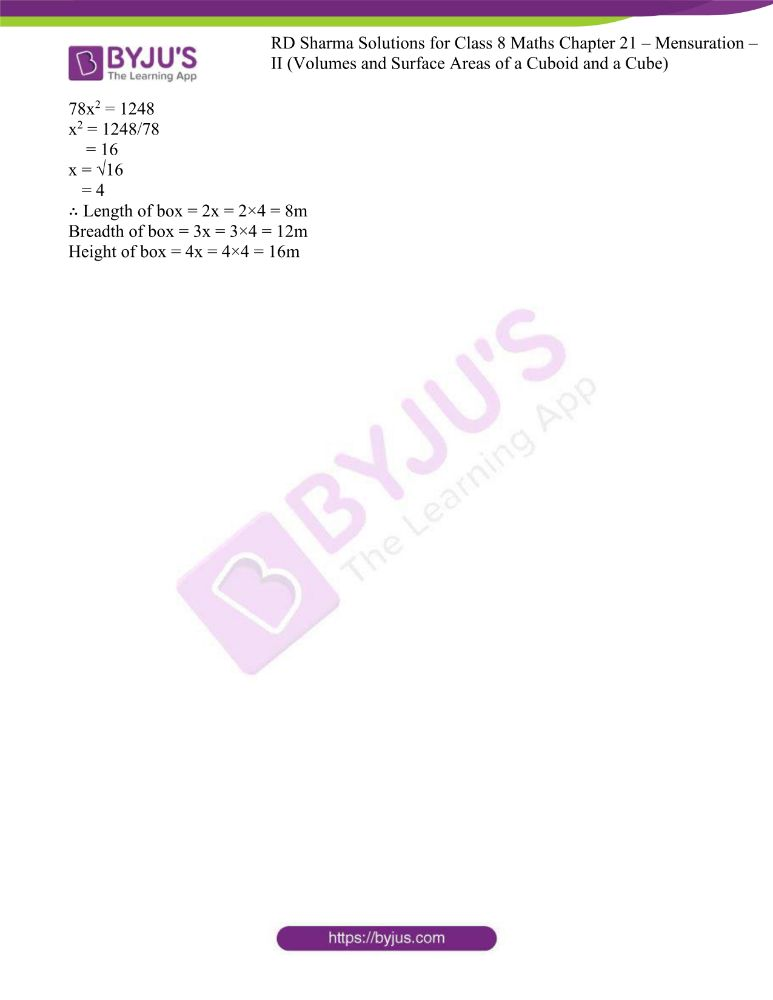### Access answers to Maths RD Sharma Solutions For Class 8 Chapter 21 Mensuration – II (Volumes and Surface Areas of a Cuboid and a cube)

EXERCISE 21.1 PAGE NO: 21.8

1. Find the volume of a cuboid whose
(i) length = 12 cm, breadth = 8 cm, height = 6 cm
(ii) length = 1.2 m, breadth = 30 cm, height = 15 cm
(iii) length = 15 cm, breadth = 2.5 dm, height = 8 cm.

Solution:

(i) The given details are:

Length of a cuboid = 12 cm

Breadth of a cuboid = 8 cm

Height of a cuboid = 6 cm

By using the formula

Volume of a cuboid = length × breadth × height

= 12 × 8 ×6

= 576 cm3

(ii) The given details are:

Length of a cuboid = 1.2 m = 120 cm

Breadth of a cuboid = 30 cm

Height of a cuboid = 15 cm

By using the formula

Volume of a cuboid = length × breadth × height

= 120 × 30 × 15

= 54000 cm3

(iii) The given details are:

Length of a cuboid = 15 cm

Breadth of a cuboid = 2.5 dm = 25 cm

Height of a cuboid = 8 cm

By using the formula

Volume of a cuboid = length × breadth × height

= 15 × 25 × 8

= 3000 cm3

2. Find the volume of a cube whose side is
(i) 4 cm (ii) 8 cm
(iii) 1.5 dm (iv) 1.2 m
(v) 25 mm

Solution:

(i) Given details are,

Side of cube = 4 cm

Volume of cube = (side) 3

= 43 = 64 cm3

(ii) Given details are,

Side of cube = 8 cm

Volume of cube = (side) 3

= 83 = 512 cm3

(iii) Given details are,

Side of cube = 1.5 dm

Volume of cube = (side) 3

= 1.53 = 3.375 dm3 = 3375 cm3

(iv) Given details are,

Side of cube = 1.2 m

Volume of cube = (side) 3

= 1.23 = 1.728 m3

(v) Given details are,

Side of cube = 25 mm

Volume of cube = (side) 3

= 253 = 15625 mm3= 15.625 cm3

3. Find the height of a cuboid of volume 100 cm3, whose length and breadth are 5 cm and 4 cm respectively.

Solution:

Given details are,

Volume of a cuboid = 100 cm3

Length of a cuboid = 5 cm

Breadth of a cuboid = 4 cm

Let height of cuboid be ‘h’ cm

We know that, l × b × h = 100cm

h = 100/( l × b)

= 100/(5×4)

= 5cm

4. A cuboidal vessel is 10 cm long and 5 cm wide. How high it must be made to hold 300 cm3 of a liquid?

Solution:

Given details are,

Volume of a liquid in the vessel = 300 cm3

Length of a cuboidal vessel = 10 cm

Breadth of a cuboidal vessel = 5 cm

Let height of cuboidal vessel be ‘h’ cm

We know that, l × b × h = 300 cm3

h = 300/(l × b)

= 300/(10×5)

= 6cm

5. A milk container is 8 cm long and 50 cm wide. What should be its height so that it can hold 4 litres of milk?

Solution:

Given details are,

Volume = 4 litres = 4000 cm3

Length of a milk container = 8 cm

Breadth of a milk container = 50 cm

Let height of milk container be ‘h’ cm

We know that, l × b × h = 4000 cm3

h = 4000/ (l × b)

= 4000/ (50×8)

= 10cm

6. A cuboidal wooden block contains 36 cm3 wood. If it be 4 cm long and 3 cm wide, find its height.

Solution:

Given details are,

Volume of wooden block = 36 cm3

Length of the wooden block = 4 cm

Breadth of a wooden block = 3 cm

Let height of wooden block be ‘h’ cm

We know that, l × b × h = 36 cm3

h = 36/( l × b)

= 36/(4×3)

= 3cm

7. What will happen to the volume of a cube, if its edge is

(i) halved (ii) trebled?

Solution:

Let us consider edge of a cube be ‘a’ cm

Volume of a cube will be ‘a3’cm

(i) When halved

Edge = a/2

Volume = (a/2)3 = a3/23 = a3/8 = 1/8times

(ii) When trebled

Edge = 3a

Volume = (3a)3 = 27a3 = 27times

8. What will happen to the volume of a cuboid if its:
(i) Length is doubled, height is same and breadth is halved?
(ii) Length is doubled, height is doubled and breadth is same?

Solution:

Let us consider,

Length of a cuboid be ‘l’

Breadth of a cuboid be ‘b’

Height of a cuboid be ‘h’

So, Volume of a cuboid = l × b × h

Now,

(i) Length of a cuboid becomes = 2l

Height = h

Volume of cuboid = 2l × b/2 × h = l × b × h (remains same)

(ii) Length of a cuboid becomes = 2l

Height = 2h

Volume of cuboid = 2l × b × 2h = 4lbh (four times)

9. Three cuboids of dimensions 5 cm × 6cm × 7cm, 4cm × 7cm × 8cm and 2 cm × 3 cm × 13 cm are melted and a cube is made. Find the side of cube.

Solution:

Given details are,

Volume of First cuboid = 5 × 6 × 7 = 210 cm3

Volume of second cuboid = 4 × 7 × 8 = 224 cm3

Volume of third cuboid = 2 × 3 × 13 = 78 cm3

So, Volume of a cube = 210 + 224 + 78 = 512 cm3

Let side of a cube be ‘a’

a3 = 512

∴ a = 8 cm

10. Find the weight of solid rectangular iron piece of size 50 cm×40cm × 10 cm, if 1 cm3 of iron weights 8 gm.

Solution:

Given details are,

Dimension of rectangular iron piece = 50cm × 40cm × 10cm

Volume of solid rectangular = 50 × 40 × 10 = 20000 cm3

Weight of 1 cm3 iron = 8 gm.

∴ Weight of 20000 cm3 iron = 8 × 20000

= 160000 gm.

= 160 kg

11. How many wooden cubical blocks of side 25 cm can be cut from a log of wood of size 3 m by 75 cm by 50 cm, assuming that there is no wastage?

Solution:

Given details are,

Dimensions of log of wood = 3m × 75cm × 50cm

Side of cubical block = 25cm

We know that,

Number of cubical block that can be made from wooden log =

Volume of wooden block / volume of cubical block

= (300 × 75 × 50) / (25 × 25 × 25)

= 72 blocks

12. A cuboidal block of silver is 9 cm long, 4 cm broad and 3.5 cm in height. From it, beads of volume 1.5 cm3 each are to be made. Find the number of beads that can be made from the block.

Solution:

Given details are,

Length of a cuboidal block of silver = 9cm

Height = 3.5cm

Volume of a cuboid = l × b × h

= 9 × 4 × 3.5 = 126cm3

So, Number of beads of volume 1.5cm3 that can be made from the block =

Volume of silver block/volume of one bead

= 126cm3/1.5cm3

13. Find the number of cuboidal boxes measuring 2 cm by 3 cm by 10 cm which can be stored in a carton whose dimensions are 40 cm, 36 cm, and 24 cm.

Solution:

Given details are,

Dimensions of cuboidal boxes is = 2cm × 3cm × 10 cm

Dimensions of carton is = 40cm × 36cm × 24cm

So,

Number of boxes that can be stored in carton = volume of carton / volume of one box

= (40 × 36 × 24) / (2 × 3 × 10)

= 576 cuboidal boxes

14. A cuboidal block of solid iron has dimensions 50 cm, 45 cm, and 34 cm, how many cuboids of size 5 cm by 3 cm by 2 cm can be obtained from this block? Assume cutting causes no wastage.

Solution:

Given details are,

Dimensions of cuboidal block of iron is = 50cm × 45cm × 34cm

Size of small cuboids cutting from it is = 5cm × 3cm × 2cm

So,

Number of small cuboids that can be cut =

Volume of large iron cuboid/ volume of small cuboid

= (50 × 45 × 34) / (5 × 3 × 2)

= 2550 cuboidal blocks

15. A cube A has side thrice as long as that of cube B. What is the ratio of the volume of cube A to that of cube B?

Solution:

Given details are,

Let side of cube B be ‘x’ cm

Then, side of cube A = 3x cm

So now,

Ratio = volume of cube A / volume of cube B

= (3x)3 / (x)3

= 27x3/ x3 = 27/1 = 27:1

16. An ice-cream brick measures 20 cm by 10 cm by 7 cm. How many such bricks can be stored in deep fridge whose inner dimensions are 100 cm by 50 cm by 42 cm?

Solution:

Given details are,

Dimensions of ice cream brick = 20 cm × 10cm × 7cm

Dimensions of fridge is = 100 cm × 50cm × 42 cm

So,

Number of bricks that can be put in fridge = volume of fridge / volume of one ice brick

= (100 × 50 × 42) / (20 × 10 × 7)

= 150 ice cream bricks

17. Suppose that there are two cubes, having edges 2 cm and 4 cm, respectively. Find the volumes V1 and V2 of the cubes and compare them.

Solution:

Given details are,

Edge of one cube a1 = 2 cm

Edge of second cube a2 = 4 cm

So, volume v1 = 23 = 8cm3

Volume v2 = 43 = 64cm3

v2 = 8v1

18. A tea-packet measures 10 cm × 6 cm × 4 cm. How many such tea-packets can be placed in a cardboard box of dimensions 50 cm × 30cm × 0.2 m?

Solution:

Given details are,

Dimensions of tea packet = 10 cm × 6 cm × 4cm

Dimension of cardboard box = 50cm × 30cm × 0.2 m

So,

Number of tea packets can be put in cardboard box =

Volume of cardboard box / volume of tea packet

= (50 × 30 × 20) / (10 × 6 × 4)

= 125 tea packets

19. The weight of a metal block of size 5 cm by 4 cm by 3 cm is 1 kg. Find the weight of a block of the same metal of size 15 cm by 8 cm by 3 cm.

Solution:

Given details are,

Dimensions of metal block = 5cm × 4cm × 3cm

Weight of block = 1 kg

Volume of box = 5×4×3 = 60 cm3

Dimension of new block = 15cm × 8cm × 3cm

Volume of new box = 15 × 8 × 3 = 360 cm3

We know that,

60cm3 = 1kg

360 cm3 = 6 × 60 cm3

= 6 × 1

= 6 kg

20. How many soap cakes can be placed in a box of size 56 cm × 0.4 m × 0.25 m, if the size of a soap cake is 7 cm × 5cm × 2.5 cm?

Solution:

Given details are,

Dimensions of box = 56cm × 0.4m × 0.25m

Dimensions of soap cake = 7cm × 5cm × 2.5cm

So,

Number of soap cakes that can be placed in box = volume of box / volume of soap cake

= (56 × 40 × 25) / (7 × 5 × 2.5)

= 640 soap cakes

21. The volume of a cuboidal box is 48 cm3. If its height and length are 3 cm and 4 cm respectively, find its breadth.

Solution:

Given details are,

Volume of a cuboidal box = 48 cm3

Length of a cuboidal box = 4 cm

Height of a cuboidal box = 3 cm

Let breadth of wooden block be ‘b’ cm

We know that, l × b × h = 48 cm3

b = 48/( l × h)

= 48/(4×3)

= 4cm

EXERCISE 21.2 PAGE NO: 21.15

1.  Find the volume in cubic metres (cu. m) of each of the cuboids whose dimensions are:
(i) length = 12 m, breadth = 10 m, height = 4.5 m
(ii) length = 4 m, breadth = 2.5m, height = 50 cm
(iii) length = 10m, breadth = 25 dm, height = 25 cm.

Solution:

(i) Given details are,

Length of a cuboid = 12 m

Breadth of a cuboid = 10m

Height of a cuboid = 4.5 m

By using the formula

Volume of cuboid = l × b × h

= 12 × 10 × 4.5

= 540 m3

(ii) Given details are,

Length of a cuboid = 4 m

Breadth of a cuboid = 2.5 m

Height of a cuboid = 50 cm = 0.50m

By using the formula

Volume of a cuboid = l × b × h

= 4 × 2.5 × 0.50

= 5 m3

(iii) Given details are,

Length of a cuboid = 10m

Breadth of a cuboid = 25 dm = 2.5 m

Height of a cuboid = 25 cm = 0.25 m

By using the formula

Volume of a cuboid = l × b × h

= 10 × 2.5 × 0.25

= 6.25 m3

2. Find the volume in cubic decimetre of each of the cubes whose side is
(i) 1.5 m

(ii) 75cm
(iii) 2 dm 5 cm

Solution:

(i) Given details are,

Side of cube = 1.5m = 15 dm

So, Volume of cube = 153 = 3375 dm3

(ii) Given details are,

Side of cube = 75cm = 7.5 dm

So, Volume of cube = 7.53 = 421.875 dm3

(iii) Given details are,

Side of cube = 2dm 5cm = 2.5 dm

So, Volume of cube = 2.53 = 15.625 dm3

3. How much clay is dug out in digging a well measuring 3 m by 2 m by 5 m?

Solution:

Given details are,

Dimensions of well = 3m × 2m × 5m

So,

Volume of clay dug out from well is = l × b × h

= 3 × 2 × 5

= 30 m3

4. What will be the height of a cuboid of volume 168 m3, if the area of its base is 28 m2?

Solution:

Given details are,

Volume of a cuboid = 168 m3

Area of base = l × b = 28m2

Let height of cuboid be ‘h’ m

We know that,

Volume = l × b × h

h = volume/ l × b

= 168/28

= 6m

∴ Height of cuboid is 6 m

5. A tank is 8 m long, 6 m broad and 2 m high. How much water can it contain?

Solution:

Given details are,

Dimensions of a tank = 8m × 6m × 2m

We know that,

Volume of tank = l × b × h

= 8 × 6 × 2

= 96 m3

= 96000 litres

∴ The tank can contain 96000 litres of water.

6. The capacity of a certain cuboidal tank is 50000 litres of water. Find the breadth of the tank, if its height and length are 10 m and 2.5 m respectively.

Solution:

Given details are,

Capacity (volume) of cuboidal tank is = 50000 litre = 50 m3

Height of tank = 10 m

Length of tank = 2.5 m

Let breadth of tank be ‘b’ m

We know that,

Volume = l × b × h

b = volume / (l × h)

= 50/(10×2.5)

= 2m

∴ Breadth of tank is 2m

7. A rectangular diesel tanker is 2m long, 2m wide and 40cm deep. How many litres of diesel can it hold?

Solution:

Given details are,

Length of a tanker = 2m

Breadth of a tanker = 2m

Height of a tanker = 40cm = 0.4m

So, Dimensions of rectangular diesel tank = 2m × 2m × 0.4m

Volume of tank (amount of diesel it can hold) = l × b × h

= 2 × 2 × 0.4

= 1.6m3

= 1600 litres

∴ A rectangular diesel tanker can hold 1600 litres of diesel.

8. The length, breadth and height of a room are 5 m, 4.5 m and 3 m respectively. Find the volume of the air it contains.

Solution:

Given details are,

Length of a room = 5m

Breadth of a room = 4.5m

Height of a room = 3m

So, Dimensions of a room are = 5m × 4.5m × 3m

Volume of air = l × b × h

= 5 × 4.5 × 3

= 67.5m3

∴ The room contains 67.5m3 volume of the air.

9. A water tank is 3 m long, 2 m broad and 1 m deep. How many litres of water can it hold?

Solution:

Given details are,

Length of water tank = 3m

Breadth of water tank = 2m

Height of water tank = 1m

So, Dimensions of water tank is = 3m × 2m × 1m

Volume the water tank can hold = l × b × h

= 3 × 2 × 1

= 6m3

= 6000 litres

∴ The water tank can hold 6000 litres of water.

10. How many planks each of which is 3 m long, 15 cm broad and 5 cm thick can be prepared from a wooden block 6 m long, 75 cm broad and 45 cm thick?

Solution:

Given details are,

Dimensions of one plank = 3m × 15cm × 5cm = 300cm × 15cm × 5cm

Dimensions of wooden block = 6m × 75cm × 45cm = 600cm × 75cm × 45cm

We know that,

Number of planks that can be prepared = volume of wooden block / volume of one plank

= (600 × 75 × 45) / (300 × 15 × 5)

= 90 planks

∴ 90 planks are required to prepare the block.

11. How many bricks each of size 25 cm × 10 cm × 8 cm will be required to build a wall 5 m long, 3 m high and 16 cm thick, assuming that the volume of sand and cement used in the construction is negligible?

Solution:

Given details are,

Size of one brick = 25cm × 10cm × 8cm

Dimensions of wall = 5m × 3m × 16cm = 500 cm × 300 cm × 16cm

We know that,

Number of bricks required to build a wall = volume of wall / volume of one brick

= (500×300×16) / (25×10×8)

= 1200 bricks

∴ 1200 bricks are required to build the wall.

12. A village, having a population of 4000, required 150 litres water per head per day. It has a tank which is 20 m long, 15 m broad and 6 m high. For how many days will the water of this tank last?

Solution:

Given details are,

Population of village = 4000

Dimensions of water tank = 20m × 15m × 6m

Water required per head per day = 150 litres

Total requirement of water per day = 150 × 4000 = 600000 litres

Volume of water tank = l × b × h

= 20 × 15 × 6

= 1800m3

= 1800000 litres

We know that,

Number of days water last in the tank = volume of tank / total requirement

= 1800000/600000

= 3 days

∴ Water in the tank last for 3 days.

13. A rectangular field is 70 m long and 60 m broad. A well of dimensions 14 m × 8 m × 6 m is dug outside the field and the earth dug-out from this well is spread evenly on the field. How much will the earth level rise?

Solution:

Given details are,

Dimensions of rectangular field = 70m × 60m

Dimensions of well = 14m × 8m × 6m

Amount of earth dug out from well (volume) = l × b × h

= 14 × 8 × 6 = 672m3

We know that,

Rise in earth level = dimensions of rectangular field / amount of earth dug up

= (70×60) / 672

= 0.16m

= 16cm

∴ Rise in earth level on a rectangular field is 16cm.

14. A swimming pool is 250 m long and 130 m wide. 3250 cubic metres of water is pumped into it. Find the rise in the level of water.

Solution:

Given details are,

Dimensions of swimming pool = 250 m × 130m

Volume of water pumped in it = 3250 m3

We know that,

Rise in water level in pool = volume of water pumped / dimensions of swimming pool

= 3250/(250×130)

= 0.1m

∴ Rise in level of water is 0.1m

15. A beam 5 m long and 40 cm wide contains 0.6 cubic metre of wood. How thick is the beam?

Solution:

Given details are,

Length of beam = 5 m

Width of beam = 40 cm = 0.4 m

Volume of wood in beam = 0.6 m3

Let thickness of beam be ‘h’ m

We know that,

Volume = l × b × h

h = volume/(l × b)

= 0.6/(5×0.4)

= 0.3m

∴ Thickness of the beam is 0.3m

16. The rainfall on a certain day was 6 cm. How many litres of water fell on 3 hectares of field on that day?

Solution:

Given details are,

Area of field = 3 hectare = 3×10000 m2 = 30000 m2

Depth of water on the field = 6cm = 6/100 = 0.06m

Volume of water = area of field × depth of water

= 30000 × 0.06

= 1800 m3

We know that 1m3 = 1000 litre

So, 1800 m3 = 1800 × 1000

= 18 × 105 litre

∴ 18 × 105 litres of water fell on 3hectares of field.

17. An 8 m long cuboidal beam of wood when sliced produces four thousand 1 cm cubes and there is no wastage of wood in this process. If one edge of the beam is 0.5 m, find the third edge.

Solution:

Given details are,

Length of cuboidal beam = 8m

One edge of beam = 0.5m

Let the third edge of beam be ‘h’ m

Number of cubes of side 1cm (.01 m) produced = 4000

We know that,

Volume of beam = volume of each cube × no. of cubes

8 × 0.5 × h = 4000 × (0.01)3

h = 0.004/4

= 0.001m

∴ Length of third edge is 0.001 m

18. The dimensions of a metal block are 2.25 m by 1.5 m by 27 cm. It is melted and recast into cubes, each of the side 45 cm. How many cubes are formed?

Solution:

Given details are,

Dimensions of metal block = 2.25m × 1.5m × 27cm = 2.25m × 1.5m × 0.27m

Side of each cube formed = 45cm = 0.45 m

We know that,

Number of cubes can formed = volume of metal block / volume of one cube

= (2.25×1.5×0.27) /(0.45×0.45×0.45)

= 0.91125 / 0.091125

= 10 cubes

∴ 10 cubes are formed.

19. A solid rectangular piece of iron measures 6 m by 6 cm by 2 cm. Find the weight of this piece, if 1 cm3 of iron weighs 8 gm.

Solution:

Given details are,

Dimensions of solid rectangular piece = 6m × 6cm × 2cm

Volume of rectangular iron = 600cm × 6cm × 2cm = 7200cm3

Weight of 1cm3 iron = 8 gm.

So, weight of 7200cm3 = 7200 × 8

= 57600 gm.

= 57.6 kg

∴ The weight of the piece is 57.6 kg

20. Fill in the blanks in each of the following so as to make the statement true :
(i) 1 m3 = ……….. cm3
(ii) 1 litre = ……. cubic decimetre
(iii) 1 kl = ………… m3
(iv) The volume of a cube of side 8 cm is …….
(v) The volume of a wooden cuboid of length 10 cm and breadth 8 cm is 4000 cm3. The height of the cuboid is …. cm.
(vi) 1 cu. dm = …….. cu. mm
(vii) 1 cu. km = ……… cu. m
(viii) 1 litre = ……cu. cm
(ix) 1 ml = ……. cu. cm
(x) 1 kl = …… cu. dm = ……….. cu. cm.

Solution:

(i) 1 m3 = 1 × (100×100×100) = 106cm3 (since 1m = 100cm)

(ii) 1 litre = 1000cm3 = 1000 × (0.1×0.1×0.1) dm3 = 1dm3 (since 1cm = 0.1dm)

(iii) 1 kl = 1000 litre = 1m3 (since 1m3 = 1000 litre)

(iv) The volume of a cube of side 8 cm is ……

We know that, side of a cube = 8cm

So, volume of cube = 83 = 512cm3

(v) The volume of a wooden cuboid of length 10 cm and breadth 8 cm is 4000 cm3. The height of the cuboid is …. cm.

Given, volume of cuboid = 4000 cm3

Length of cuboid = 10cm

We know that volume = l × b × h

h = volume / (l × b)

= 4000 / (10×8)

= 50cm

(vi) 1 cu. dm = 1dm3 = 1× (10×10×10) = 103cm3 (since 1dm = 10cm)

103× (10×10×10) = 106 mm3 (since 1cm = 10mm)

(vii) 1 cu. km = 1000×1000×1000 = 109m3 (since 1km = 1000m)

(viii) 1 litre = 1000 cm3 = 103cm3

(ix) 1 ml = 1/1000 litre = 1/1000 × 1000 = 1cm3 (since 1ml = 1/1000 litre)

(x) 1 kl = 1 × 1000 litre = 1m3 = 1 × (10×10×10) dm3 (since 1m = 10dm)

1 kl = 1000 dm3 = 103 dm3 = 1000 × 1000 = 106 cm3

EXERCISE 21.3 PAGE NO: 21.22

1. Find the surface area of a cuboid whose

(i) length = 10 cm, breadth = 12 cm, height = 14 cm
(ii) length = 6 dm, breadth = 8 dm, height = 10 dm
(iii) length = 2m, breadth = 4 m, height = 5 m
(iv) length = 3.2 m, breadth = 30 dm, height = 250 cm.

Solution:

(i) Given details are,

Length of a cuboid = 10 cm

Breadth of a cuboid = 12 cm

Height of a cuboid = 14 cm

We know that,

Surface area of cuboid = 2 (lb + bh + hl) cm2

= 2 (10×12 + 12×14 + 14×10)

= 2 (120 + 168 + 140)

= 2 (428)

= 856 cm2

(ii) Given details are,

Length of a cuboid = 6 dm

Breadth of a cuboid = 8 dm

Height of a cuboid = 10 dm

We know that,

Surface area of cuboid = 2 (lb + bh + hl) cm2

= 2 (6×8 + 8×10 + 10×6)

= 2 (48 + 80 + 60)

= 2 (188)

= 376 dm2

(iii) Given details are,

Length of a cuboid = 2m

Breadth of a cuboid = 4m

Height of a cuboid = 5m

We know that,

Surface area of cuboid = 2 (lb + bh + hl) cm2

= 2 (2×4 + 4×5 + 5×2)

= 2 (8 + 20 + 10)

= 2 (38)

= 76 m2

(iv) Given details are,

Length of a cuboid = 3.2 m= 32 dm

Breadth of a cuboid = 30 dm

Height of a cuboid = 250 cm= 25 dm

We know that,

surface area of cuboid = 2 (lb + bh + hl) cm2

= 2 (32×30 + 30×25 + 25×32)

= 2 (960 + 750 + 800)

= 2 (2510)

= 5020 dm2

2. Find the surface area of a cube whose edge is
(i) 1.2 m
(ii) 27 cm
(iii) 3 cm
(iv) 6 m
(v) 2.1 m

Solution:

(i) Given,

Edge of cube = 1.2 m

We know that,

Surface area of cube = 6 × side2

= 6 × 1.22

= 6 × 1.44

= 8.64 m2

(ii) Given,

Edge of cube = 27 cm

We know that,

Surface area of cube = 6 × side2

= 6 × 272

= 6 × 729

= 4374 cm2

(iii) Given,

Edge of cube = 3 cm

We know that,

Surface area of cube = 6 × side2

= 6 × 32

= 6 × 9

= 54 cm2

(iv) Given,

Edge of cube = 6 m

We know that,

Surface area of cube = 6 × side2

= 6 × 62

= 6 × 36

= 216 m2

(v) Given,

Edge of cube = 2.1 m

We know that,

Surface area of cube = 6 × side2

= 6 × 2.12

= 6 × 4.41

= 26.46 m2

3. A cuboidal box is 5 cm by 5 cm by 4 cm. Find its surface area.

Solution:

Given details are,

Dimensions of cuboidal box = 5cm × 5cm × 4cm

We know that,

Surface area of cuboid = 2 (lb + bh + hl) cm2

= 2 (5×5 + 5×4 + 4×5)

= 2 (25 + 20 + 20)

= 2 (65)

= 130 cm2

4. Find the surface area of a cube whose volume is
(i) 343 m3
(ii) 216 dm3

Solution:

(i) Given details are,

Volume of cube = 343 m3

Side of cube, a = 3√(343) = 7m

We know that,

Surface area of cube = 6 × side2

= 6 × 72

= 6 × 49

= 294 m2

(ii) Given details are,

Volume of cube = 216 dm3

Side of cube a = 3√(216) = 6dm

We know that,

Surface area of cube = 6 × side2

= 6 × 62

= 6 × 36

= 216 dm2

5. Find the volume of a cube whose surface area is

(i) 96 cm2
(ii) 150 m2

Solution:

(i) Given details are,

Surface area of cube = 96 cm2

6 × side2 = 96cm2

Side2 = 96/6

= 16

Side = √16 = 4cm

∴ Volume of a cube = 43 = 64cm3

(ii) Given details are,

Surface area of cube = 150 m2

6 × side2 = 150cm2

Side2 = 150/6

= 25

Side = √25 = 5cm

∴ Volume of a cube = 53 = 125m3

6. The dimensions of a cuboid are in the ratio 5: 3: 1 and its total surface area is 414 m2. Find the dimensions.

Solution:

Given details are,

Ratio of dimensions of a cuboid = 5:3:1

Total surface area of cuboid = 414 m2

The dimensions are = 5x × 3x × x

Surface area of cuboid = 414 m2

We know that,

Surface area of cuboid = 2 (lb + bh + hl) cm2

2 (lb + bh + hl) cm2 = 414

2 (15x2 + 3x2 + 5x2) = 414

2 (23x2) = 414

46x2 = 414

x2 = 414/46

= 9

x = √9

= 3

∴ Dimensions are,

5x = 5 (3) = 15m

3x = 3 (3) = 9m

x = 3m

7. Find the area of the cardboard required to make a closed box of length 25 cm, 0.5 m and height 15 cm.

Solution:

Given details are,

Dimensions of closed box = 25cm × 0.5m × 15cm = 25cm × 50cm × 15cm

We know that,

Area of cardboard required = 2 (lb + bh + hl) cm2

= 2 (25×50 + 50×15 + 15×25)

= 2 (1250 + 750 + 375)

= 2 (2375)

= 4750 cm2

8. Find the surface area of a wooden box whose shape is of a cube, and if the edge of the box is 12 cm.

Solution:

Given details are,

Edge of a cubic wooden box = 12 cm

We know that,

Surface area of cubic wooden box = 6 × side2

= 6 × 122

= 6 × 144

= 864 cm2

9. The dimensions of an oil tin are 26 cm× 26 cm× 45 cm. Find the area of the tin sheet required for making 20 such tins. If 1 square metre of the tin sheet costs Rs. 10, find the cost of tin sheet used for these 20 tins.

Solution:

Given details are,

Dimensions of oil tin = 26cm × 26cm × 45cm

Then,

Area of tin sheet required for making one oil tin = total surface area of oil tin

= 2 (lb + bh + hl) cm2

= 2 (26×26 + 26×45 + 45×26)

= 2 (676 + 1170 + 1170)

= 2 (3016)

= 6032 cm2

Area of tin sheet required for 20 oil tins = 20 × 6032

= 120640 cm2

= 12.064 m2

Given, Cost of 1 m2 tin sheet = Rs 10

So, Cost of 12.064 m2 tin sheet = 10 × 12.064

= Rs 120.60

10. A classroom is 11 m long, 8 m wide and 5 m high. Find the sum of the areas of its floor and the four walls (including doors, windows etc.)

Solution:

Given details are,

Dimensions of class room = 11m × 8m × 5m

Where, Length = 11m, Breadth = 8m, Height = 5m

We know,

Area of floor = length × breadth

= 11 × 8

= 88 m2

Area of four walls (including doors & windows) = 2 (lh + bh) cm2

= 2 (11×5 + 8×5)

= 2 (55 + 40)

= 2 (95)

= 190m2

∴ Sum of areas of floor and four walls = area of floor + area of four walls

= 88 + 190

= 278 m2

11. A swimming pool is 20 m long 15 m wide and 3 m deep. Find the cost of repairing the floor and wall at the rate of Rs. 25 per square metre.

Solution:

Given details are,

Dimensions of swimming pool are = 20m × 15m ×3m

Where, Length = 20m , Breadth = 15m , Height = 3m

We know,

Area of floor = length × breadth

= 20 × 15

= 300 m2

Area of walls of swimming pool = 2 (lh + bh) cm2

= 2 (20×3 + 15×3)

= 2 (60 + 45)

= 2 (105)

= 210m2

Sum of areas of floor and four walls = area of floor + area of walls

= 300 + 210

= 510 m2

Given, Cost for repairing 1m2 area = Rs 25

∴ Cost for repairing 510 m2 = 510 × 25

= Rs 12750

12. The perimeter of a floor of a room is 30 m and its height is 3 m. Find the area of four walls of the room.

Solution:

Given details are,

Height of floor = 3m

Perimeter of floor = 30m

So, perimeter = 30

2(l+b) = 30

l+b = 30/2

l+b = 15m

∴ Area of four walls of room = 2 (lh + bh) m2

= 2h (l+b)

= 2 (3) (15)

= 90m2

13. Show that the product of the areas of the floor and two adjacent walls of a cuboid is the square of its volume.

Solution:

Let us consider length of cuboid as = l cm

Let us consider breadth of cuboid as = b cm

Let us consider height of cuboid as = h cm

We know,

Area of floor = l × b = lb cm2

Then,

Product of areas of two adjacent walls = (l×h) × (b×h) = lbh2 cm4

Product of areas of floor and two adjacent walls = lb × lbh2 cm6

= l2 × b2 × h2 cm6

= (lbh)2 cm6

We know, volume of cuboid = lbh cm

Hence, areas of the floor and two adjacent walls of a cuboid is the square of its volume.

14. The walls and ceiling of a room are to be plastered. The length, breadth nad height of the room are 4.5 m, 3 m and 350 cm, respectively. Find the cost of plastering at the rate of Rs. 8 per square metre.

Solution:

Given details are,

Length of room = 4.5m

Height of wall = 350cm = 350/100 = 3.5m

Area of ceiling = l × b

= 4.5 × 3

= 13.5 m2

Area of walls = 2 (lh + bh) m2

= 2 (4.5×3.5 + 3×3.5)

= 2 (15.75 + 10.5)

= 52.5 m2

Sum of Area of ceiling + area of walls = 13.5m2 + 52.5m2

= 66m2

Given, Cost for plastering 1m2 area = Rs 8

∴ Cost for plastering 66 m2 area = 66 × 8 = Rs 528

15. A cuboid has total surface area of 50 m2 and lateral surface area is 30 m2. Find the area of its base.

Solution:

Given details are,

Total surface area of cuboid = 50 m2

Lateral surface area of cuboid = 30 m2

Total Surface area = 2 (surface area of base) + (surface area of 4 walls)

50 = 2 (surface area of base) + (lateral surface area)

50 = 2 (surface area of base) + 30

50 – 30 = 2 (surface area of base)

20 = 2 (surface area of base)

Surface area of base = 20/2

= 10 m2

∴ Area of base is 10m2

16. A classroom is 7 m long, 6 m broad and 3.5 m high. Doors and windows occupy an area of 17 m2. What is the cost of white washing the walls at the rate of Rs 1.50 per m2?

Solution:

Given details are,

Dimensions of class room = 7m × 6m × 3.5m

Where, Length = 7m, Breadth = 6m, Height = 3.5m

Area of four walls (including doors & windows) = 2 (lh + bh) m2

= 2 (7×3.5 + 6×3.5)

= 91m2

Area of four walls (without doors & windows) =

Area including doors & windows – area occupied by doors & windows

= 91 – 17 = 74 m2

Then,

Cost for white washing 1m2 area of walls = Rs 1.50

∴ Total cost for white washing the walls = 74 × 1.50 = Rs 111

17. The central hall of a school is 80 m long and 8 m high. It has 10 doors each of size 3m ×1.5m and 10 windows each of size 1.5m× 1m. If the cost of white washing the walls of the hall at the rate of Rs 1.20 per m2 is Rs 2385.60, find the breadth of the hall.

Solution:

Given details are,

Dimensions of central hall of a school = Length = 80 m , height = 8m

Let breadth of hall be ‘b’ m

So,

Area of each door = 3m × 1.5m = 4.5m2

Area of 10 doors = 10 × 4.5 = 45m2

Area of each window = 1.5m × 1m = 1.5 m2

Area of 10 windows = 10 × 1.5 = 15m2

Area occupied by doors and windows = 45 + 15 = 60 m2

Area of the walls of the hall including doors and windows = 2 (lh + bh) m2

= 2 (80×8 + b×8)

= 2(640+8b) m2

Then,

Area of only walls = area of walls including doors & windows – area occupied by doors & windows

= 2(640+8b) – 60

= 1280 + 16b – 60

= (1220 + 16b) m2

Given, Total cost for white washing = Rs 2385.60

Rate of white washing = Rs 1.20 per m2

So,

Total cost = Rate × (areas of walls only)

2385.60 = 1.20 × (1220 + 16b)

2385.60 / 1.20 = (1220 + 16b)

1988 = 1220 + 16b

16b = 1988 – 1220

= 768

b = 768/16

= 48

∴ Breadth of hall is 48 m

EXERCISE 21.4 PAGE NO: 21.30

1. Find the length of the longest rod that can be placed in a room 12 m long, 9 m broad and 8 m high.

Solution:

Given details are,

Length of room = 12 m

Height of room = 8m

So,

Length of longest rod that can be placed in room = diagonal of room (cuboid)

= √(l2 + b2 + h2)

= √(122 + 92 + 82)

= √(144+81+64)

= √(289)

= 17m

2. If V is the volume of a cuboid of dimensions a, b, c and S is its surface area, then prove that 1/V = 2/S (1/a + 1/b + 1/c)

Solution:

Let us consider,

V = volume of cuboid

S = surface area of cuboid

Dimensions of cuboid = a, b, c

So,

S = 2 (ab + bc + ca)

V = abc

S/V = 2 (ab + bc + ca) / abc

= 2[(ab/abc) + (bc/abc) + (ca/abc)]

= 2 (1/a + 1/b + 1/c)

1/V = 2/S (1/a + 1/b + 1/c)

Hence proved.

3. The areas of three adjacent faces of a cuboid are x, y, and z. If the volume is V, prove that V2 = xyz.

Solution:

Let us consider,

Areas of three faces of cuboid as x,y,z

So, Let length of cuboid be = l

Breadth of cuboid be = b

Height of cuboid be = h

Let, x = l×b

y = b×h

z = h×l

Else we can write as

xyz = l2 b2 h2….. (i)

If ‘V’ is volume of cuboid = V = lbh

V2 = l2 b2 h2 = xyz …… from (i)

∴ V2 = xyz

Hence proved.

4. A rectangular water reservoir contains 105 m3 of water. Find the depth of the water in the reservoir if its base measures 12 m by 3.5 m.

Solution:

Given details are,

Capacity of water reservoir = 105 m3

Length of base of reservoir = 12 m

Width of base = 3.5 m

Let the depth of reservoir be ‘h’ m

l × b × h = 105

h = 105 / (l × b)

= 105 / (12×3.5)

= 105/42

= 2.5m

∴ Depth of reservoir is 2.5 m

5. Cubes A, B, C having edges 18 cm, 24 cm and 30 cm respectively are melted and moulded into a new cube D. Find the edge of the bigger cube D.

Solution:

Given details are,

Edge length of cube A = 18 cm

Edge length of cube B = 24 cm

Edge length of cube C = 30 cm

Then,

Volume of cube A = v1 = 183 = 5832cm3

Volume of cube B = v2 = 243 = 13824cm3

Volume of cube C = v3 = 303 = 27000cm3

Total volume of cube A,B,C = 5832 + 13824 + 27000 = 46656 cm3

Let ‘a’ be the length of edge of newly formed cube.

a3 = 46656

a = 3√(46656)

= 36

∴ Edge of bigger cube is 36cm

6. The breadth of a room is twice its height, one half of its length and the volume of the room is 512 cu. Dm. Find its dimensions.

Solution:

Given,

Breadth of room is twice of its height, b = 2h or h = b/2 … (i)

Breadth is one half of length, b = l/2 or l = 2b … (ii)

Volume of the room = lbh = 512 dm3 … (iii)

By substituting (i) and (ii) in (iii)

2b × b × b/2 = 512

b3 = 512

b = 3√(512)

= 8

∴ Breadth of cube = b = 8 dm

Length of cube = 2b = 2×8 = 16 dm

Height of cube = b/2 = 8/2 = 4 dm

7. A closed iron tank 12 m long, 9 m wide and 4 m deep is to be made. Determine the cost of iron sheet used at the rate of Rs. 5 per metre sheet, sheet being 2 m wide.

Solution:

Given,

Length of tank, l = 12 m

Width of tank, b = 9m

Depth of tank, h = 4m

Area of sheet required = total surface area of tank

= 2 (lb×bh×hl)

= 2 (12×9 + 9×4 + 4×12)

= 2 (108 + 36 + 48)

= 2 (192)

= 384 m2

Let length be l1

Given, b1 = 2m

l1 × b1 = 384

l1 = 384/b1

= 384/2

= 192m

∴ Cost of iron sheet at the rate of Rs 5 per metre = 5 × 192 = Rs 960

8. A tank open at the top is made of iron sheet 4 m wide. If the dimensions of the tank are 12m×8m×6m, find the cost of iron sheet at Rs. 17.50 per metre.

Solution:

Given details are,

Dimensions of tank = 12m × 8m × 6m

Where, length = 12m

Height = 6m

Area of sheet required = total surface area of tank with one top open

= l × b + 2 (l×h + b×h)

= 12 × 8 + 2 (12×6 + 8×6)

= 96 + 240

= 336 m2

Let length be l1

Given, b1 = 4m

l1 × b1 = 336

l1 = 336/b1

= 336/4

= 84m

∴ Cost of iron sheet at the rate of Rs 17.50 per metre = 17.50 × 84 = Rs 1470

9. Three equal cubes are placed adjacently in a row. Find the ratio of total surface area of the new cuboid to that of the sum of the surface areas of the three cubes.

Solution:

Given details are,

Let edge length of three equal cubes = a

Then,

Sum of surface area of 3 cubes = 3 × 6a2 = 18a2

When these cubes are placed in a row adjacently they form a cuboid.

Length of new cuboid formed = a + a + a = 3a

Height of cuboid = a

Total surface area of cuboid = 2 (lb×bh×hl)

= 2 (3a×a + a×a + a×3a)

= 2 (3a2 + a2 + 3a2)

= 2 (7a2)

= 14 a2

Total surface area of new cuboid / sum of surface area of 3 cuboids = 14/18 = 7/9 = 7:9

∴ The ratio is 7:9

10. The dimensions of a room are 12.5 m by 9 m by 7 m. There are 2 doors and 4 windows in the room; each door measures 2.5 m by 1.2 m and each window 1.5 m by 1 m. Find the cost of painting the walls at Rs. 3.50 per square metre.

Solution:

Given details are,

Dimensions of room = 12.5m × 9m × 7m

Dimensions of each door = 2.5m × 1.2m

Dimensions of each window = 1.5m × 1m

Area of four walls including doors and windows = 2 (l×h + b×h)

= 2 (12.5×7 + 9×7)

= 2 (87.5 + 63)

= 2 (150.5)

= 301 m2

Area of 2 doors and 4 windows = 2 (2.5×1.2) + 4 (1.5×1)

= 2(3) + 4 (1.5)

= 6 + 6

= 12 m2

Area of only walls = 301 – 12

= 289 m2

∴ Cost of painting the walls at the rate of Rs 3.50 per square metre = Rs (3.50 × 289) = Rs 1011.50

11. A field is 150m long and 100m wide. A plot (outside the field) 50m long and 30m wide is dug to a depth of 8m and the earth taken out from the plot is spread evenly in the field. By how much is the level of field raised?

Solution:

Given details are,

Length of field = 150m

Width of field = 100m

Area of field = 150m × 100m = 15000m2

Length of plot = 50m

Breadth of the plot = 30m

Depth = 8m

So, volume = l × b × h = 50×30×8 = 12000m3

Let raise in earth level of field on which it spread be ‘h’ metre

Volume = l × b × h

h = volume / (l × b)

= 12000 / (150×100)

= 12000 / 15000

= 0.8 m

= 80cm

∴ The level of field is raised by 80cm.

12. Two cubes, each of volume 512 cm3 are joined end to end. Find the surface area of the resulting cuboid.

Solution:

Given details are,

Volume of each cube = 512 cm3

Let length of edge of each cube be ‘a’ cm

So,

Edge, a3 = 512

a = 3√512

= 8cm

When these two cubes are joined end to end, a cuboid is formed.

Length of cuboid = 8+8 = 16 cm

Height = 8 cm

Surface area of resulting cuboid = 2 (lb + bh + hl)

= 2 (16×8 + 8×8 + 8×16)

= 2 (128 + 64 + 128)

= 2 (320)

= 640 cm2

∴ Surface area of resulting cuboid is 640cm2.

13. Three cubes whose edges measure 3 cm, 4 cm, and 5 cm respectively are melted to form a new cube. Find the surface area of the new cube formed.

Solution:

Given details are,

Edge of three cubes are = 3cm, 4cm, 5cm

Sum of volume of these cubes = 33 + 43 + 53

= 27 + 64 + 125

= 216 cm3

After these cubes are melted, a new cube is formed.

Let edge length of this new cube be ‘a’ cm

a3 = 216

a = √216

= 6cm

Edge of new cube is = 6cm

∴ Surface area of new cube = 6 × a2

= 6 × 62

= 6 × 36

= 216cm2

14. The cost of preparing the walls of a room 12m long at the rate of Rs 1.35 per square metre is Rs 340.20 and the cost of matting the floor at 85 paise per square metre is Rs 91.80. Find the height of the room.

Solution:

Given details are,

Length of room = 12 m

Let width of room be ‘b’ m

Let height of room be ‘h’ metre

Now,

Area of floor = 12×b m2 = 12b m2

Cost of matting the floor at the rate of 85 paise per square metre = Rs 91.80

12b × 0.85 = 91.80

12b = 91.80/0.85

12b = 108

b = 108/12

= 9m

Now, Breadth of room = 9m

Area of 4 walls = 2 (l×h + b×h)

= 2 (12×h + 9×h)

= 2 (12h + 9h)

= 2 (21h)

= 42h m2

Cost for preparing walls at the rate of Rs 1.35 per square metre = Rs 340.20

42h × 1.35 = 340.20

42h = 340.20/1.35

42h = 252

h = 252/42

= 6m

∴ Height of room is 6m.

15. The length of a hall is 18 m and the width 12 m. The sum of the areas of the floor and the flat roof is equal to the sum of the areas of the four walls. Find the height of the wall.

Solution:

Given details are,

Length of hall = 18m

Width of hall = 12m

Let height of hall be ‘h’ metre

Sum of area of floor and flat roof = (l×b + l×b)

= (12×18 + 12×18)

= (216+216)

= 432 m2

Sum of area of 4 walls = 2 (l×h + b×h)

= 2 (18×h + 12×h)

= 2 (18h + 12h)

= 2 (30h)

= 60h m2

Now,

Sum of area of 4 walls = sum of area of floor and flat roof

60h = 432

h = 432/60

= 7.2m

∴ Height of hall is 7.2m

16. A metal cube of edge 12 cm is melted and formed into three smaller cubes. If the edges of the two smaller cubes are 6 cm and 8 cm, find the edge of the third smaller cube.

Solution:

Given details are,

Edge of metal cube (volume) = 12cm

Edge of smaller two cubes = 6cm, 8cm

Let edge of third cube be ‘a’ cm

So,

Volume of metal cube = sum of volume of three small cubes

123 = 63 + 83 + a3

1728 = 216 + 512 + a3

a3 = 1728 – 216 – 512

= 1000

a = 3√1000

= 10cm

∴ Edge of third smaller cube is 10cm.

17. The dimensions of a cinema hall are 100 m, 50 m and 18 m. How many persons can sit in the hall, if each person required 150 m3 of air?

Solution:

Given details are,

Dimensions of cinema hall = 100m × 50m × 18m

Where,

length = 100m, breadth = 50m, height = 18 m

Each person requires = 150 m3 of air

So,

Volume of cinema hall = l × b × h

= 100 × 50 × 18

= 90000 cm3

Number of person who can sit in cinema hall = volume of hall / volume of air required by one person

= 90000 / 150

= 600

∴ 600 people can sit in the cinema hall.

18. The external dimensions of a closed wooden box are 48 cm, 36 cm and 30 cm. The box is made of 1.5 cm thick wood. How many bricks of size 6 cm× 3 cm× 0.75 cm can be put in this box?

Solution:

Given details are,

External dimensions of wooden box = 48cm × 36cm × 30cm

Dimensions of bricks = 6cm × 3cm × 0.75cm

Thickness of wood = 1.5cm

Internal dimensions of box = 48 – (2×1.5)cm × 36 – (2×1.5)cm × 30 – (2×1.5)cm

= (48–3)cm × (36-3)cm × (30-3)cm

= (45 × 33 × 27) cm

Hence,

Number of bricks can be put in box = internal volume of box / volume of one brick

= (45 × 33 × 27) / (6 × 3 × 0.75)

= 40095 / 13.5

= 2970 bricks

∴ 2970 bricks can be put in the box.

19. The dimensions of a rectangular box are in the ratio of 2: 3: 4 and the difference between the cost of covering it with sheet of paper at the rates of Rs 8 and Rs 9.50 per m2 is Rs 1248. Find the dimensions of the box.

Solution:

Given details are,

Ratio of dimensions of rectangular box = 2:3:4

Let length of box be ‘2x’ m

Let breadth of box be ‘3x’ m

Let height of box be ‘4x’ m

Area of sheet of paper required for covering it = total surface area of cuboid

= 2 (lb+bh+hl)

= 2 (2x×3x + 3x×4x + 4x×2x)

= 2 (6x2 + 12x2 + 8x2)

= 2 (26x2)

= 52 x2 m2

Cost for covering with sheet of paper at the rate of Rs 9.50 /m2 = 52x2 × 9.50

= Rs 494x2

Cost for covering with sheet of paper at the rate of Rs 8 /m2 = 52x2 × 8

= Rs 416x2

Given, the difference between the cost of covering it with sheet of paper at the rates of Rs 8 and Rs 9.50 per m2 is Rs 1248

494x2 – 416x2 = 12448

78x2 = 1248

x2 = 1248/78

= 16

x = √16

= 4

∴ Length of box = 2x = 2×4 = 8m

Breadth of box = 3x = 3×4 = 12m

Height of box = 4x = 4×4 = 16m